## Introduction

In 1972 Fritz John  published a uniqueness theorem for Nonlinear Elasticity that, until recently, was the only result of its kind. He showed that, given a stress-free reference configuration whose elasticity tensor is uniformly positive definite, there is an $$L^{\infty }$$-neighborhood of the reference configuration in the space of strains, rather than the space of deformation gradients, in which there is at most one smooth solution of the equations of equilibrium for the pure-displacement problem for a hyperelastic body.

John’s proof made use of the space of functions of Bounded Mean Oscillation, $$\operatorname{BMO}$$, a space that John and Nirenberg  had invented some ten years earlier. According to L. Nirenberg ([31, pp. 707–709]), the idea of considering functions whose mean oscillation is bounded was conceived by Fritz John. His motivation appears to have been the analysis of problems in Nonlinear Elasticity, where John had noticed that mappings with small nonlinear strain (see (5.16)) correspond to deformation gradients the are small in $$\operatorname{BMO}$$ (see  or, e.g., [43, Proposition 4.3 and Lemma 5.6]).

Subsequently, although our understanding of the space $$\operatorname{BMO}$$ and its applicability to both Harmonic Analysis and Partial Differential Equations has advanced significantly (see, e.g., the exposition by R. V. Kohn [35, p. 509-512]), the original goal of making use of $$\operatorname{BMO}$$ in problems of Elasticity has not progressed. Recently, the authors  extended John’s uniqueness result to include the mixed problem. In particular we showed that, given a smooth equilibrium solution $${\mathbf{u}}_{\mathrm{e}}$$ at which the second variation of the energy is uniformly positive, there are no other equilibrium solutions $${\mathbf{v}}_{\mathrm{e}}$$ for which the difference of the right Cauchy-Green strain tensors:

$$(\nabla {\mathbf{u}}_{\mathrm{e}})^{\mathrm{T}}\nabla {\mathbf{u}}_{ \mathrm{e}}- (\nabla {\mathbf{v}}_{\mathrm{e}})^{\mathrm{T}}\nabla { \mathbf{v}}_{\mathrm{e}}$$
(1.1)

is small in $$L^{\infty }$$. Here $$\nabla {\mathbf{u}}$$ denotes the deformation gradient: an $$n$$ by $$n$$ matrix of partial derivatives of the components of the deformation $${\mathbf{u}}:{\varOmega }\to {\mathbb{R}}^{n}$$, $$(\nabla {\mathbf{u}})^{\mathrm{T}}$$ denotes the transpose of $$\nabla {\mathbf{u}}$$, and we identify the body with the (bounded) region $${\overline{\varOmega }}\subset {\mathbb{R}}^{n}$$ that it occupies in a fixed reference configuration.

In this manuscript we extend the results obtained in [30, 43]. We note that when $${\varOmega }$$ has sufficiently smooth boundary (Lipschitz suffices), the space $$\operatorname{BMO}({\varOmega })$$ is a Banach space that is between $$L^{\infty }$$ and all of the other $$L^{p}$$-spaces, that is, for all $$p\in [1,\infty )$$,

$$L^{\infty }({\varOmega }) \subset \operatorname{BMO}({\varOmega }) \subset L^{p}({\varOmega }).$$

Specifically, $${[\kern -0.3ex] \cdot [\kern -0.3ex]}_{\operatorname{BMO}({\varOmega })}\le 2 \|\cdot \|_{L^{\infty }({\varOmega })}$$ and hence an $$\varepsilon$$-neighborhood in $$\operatorname{BMO}$$ is larger than an $$\varepsilon$$-neighborhood in $$L^{\infty }$$. Here $${[\kern -0.3ex] \cdot [\kern -0.3ex]}$$ denotes the standard seminorm on $$\operatorname{BMO}({\varOmega })$$ (see (2.3)).

We show, in particular, that the $$L^{\infty }$$-neighborhood in which there is at most one solution can be enlarged to a neighborhood in $$\operatorname{BMO}$$ for both the displacement and the mixed problem provided the equilibrium solution $${{\mathbf{u}}_{\mathrm{e}}}$$ has nonnegative principal stresses everywhere. Thus, in this case the strain difference in (1.1) need no longer be uniformly small, but instead it need only be small in the space $$\operatorname{BMO}({\varOmega })$$.

There are similar interesting results in the Calculus of Variations literature. Kristensen and Taheri [36, Sect. 6] and Campos Cordero [6, Sect. 4] (see, also, Firoozye ) have shown that, for the Dirichlet problem, if $${{\mathbf{u}}_{\mathrm{e}}}$$ is a Lipschitz-continuous solution of the equilibrium equations at which the second variation is uniformly positive, then there is a neighborhood of $$\nabla {{\mathbf{u}}_{\mathrm{e}}}$$ in $$\operatorname{BMO}$$ in which all Lipschitz mappings have energy that is greater than or equal to the energy of $${{\mathbf{u}}_{\mathrm{e}}}$$. We note that the assumptions in , in particular, are incompatible with the blowup of the energy as the Jacobian goes to zero. Recently  we have extended the results in [6, Sect. 4] to include the Neumann and mixed problems. Although our proofs are not applicable to elasticity, we have shown that given a Lipschitz-continuous solution $${{\mathbf{u}}_{\mathrm{e}}}$$ of the equilibrium equations at which the second variation is uniformly positive, there is a neighborhood of $$\nabla {{\mathbf{u}}_{\mathrm{e}}}$$ in $$\operatorname{BMO}$$ in which all mappings $${\mathbf{v}}$$ in the Sobolev space $$W^{1,1}({\varOmega };{\mathbb{R}}^{n})$$ with $$\nabla {\mathbf{v}}\in \operatorname{BMO}({\varOmega })$$ have energy that is strictly greater than the energy of $${{\mathbf{u}}_{\mathrm{e}}}$$.

We herein also establish a version of Korn’s inequality for $$\operatorname{BMO}$$. It is well-known (see, e.g., [1, 21, 28, 46]) that, for all $$p\in (1,\infty )$$, a generalized Korn inequality is valid, that is, there is a constant $$K=K(p)=K(p,n,{\varOmega })$$ such that

$$\int _{\varOmega }\big|\nabla {\mathbf{w}}({\mathbf{x}})\big|^{p}\,{ \mathrm{d}}{\mathbf{x}}\le K(p) \int _{\varOmega }\big|\nabla {\mathbf{w}}({ \mathbf{x}})+[\nabla {\mathbf{w}}({\mathbf{x}})]^{\mathrm{T}}\big|^{p}\,{ \mathrm{d}}{\mathbf{x}}$$

for all $${\mathbf{w}}\in W^{1,p}({\varOmega };{\mathbb{R}}^{n})$$ that satisfy a suitable constraint that eliminates infinitesimal rotations (e.g., $${\mathbf{w}}=\mathbf{0}$$ on $${\mathscr {D}}\subset \partial {\varOmega }$$). We show that there exists a constant $${\mathscr {K}}={\mathscr {K}}(n)$$ such that, for every nonempty, bounded open set $$U\subset {\mathbb{R}}^{n}$$,

$${[\kern -0.3ex] \nabla {\mathbf {w}}[\kern -0.3ex]}_{\operatorname{BMO}(U)} \le {\mathscr {K}} {[\kern -0.3ex] \nabla {\mathbf {w}}+(\nabla {\mathbf {w}})^{{\mathrm {T}}} [\kern -0.3ex]}_{\operatorname{BMO}(U)},$$
(1.2)

for every $${\mathbf{w}}\in W_{\operatorname{loc}}^{1,1}(U;{\mathbb{R}}^{n})$$ with $$\nabla {\mathbf{w}}\in \operatorname{BMO}(U)$$. Note that, unlike the standard Korn inequalities, which are only valid for John domains (see ) and for which the Korn constant depends on the domain, (1.2) is valid for all bounded open sets $$U$$ with a constant that is independent of $$U$$. (The lack of a constraint to eliminate infinitesimal rotations is due to the nature of the $$\operatorname{BMO}$$-seminorm. See (2.2) and (3.1).)

Before we present a more detailed description of our results, we note that there is a long history of both nonuniqueness, e.g., buckling , and uniqueness results in nonlinear elasticity. Rather than providing details here we instead refer the reader to the introductions of two recent papers concerning uniqueness [41, 43]. These papers also discuss interesting possible extensions of such results: the pure-traction problem, incompressible materials, and live loading, none of which are considered in this manuscript.

We begin in Sect. 2 with our notations. In Sect. 2.1 we then present certain consequences of the Geometric Rigidity theory of Friesecke, James, and Müller  (see, also, Conti and Schweizer  and Kohn ) that are useful in our work. In particular, a result of Lorent  as well as a result of Ciarlet and Mardare  give conditions under which the equality of two strains, $$(\nabla {\mathbf{u}})^{\mathrm{T}}\nabla {\mathbf{u}}\equiv (\nabla { \mathbf{v}})^{\mathrm{T}}\nabla {\mathbf{v}}$$, yields the equality of the underlying deformations: $${\mathbf{u}}\equiv {\mathbf{v}}$$. (This need not be true without further assumptions, even if $${\mathbf{u}}={\mathbf{v}}$$ on $$\partial {\varOmega }$$).

After reviewing certain standard properties of the space $$\operatorname{BMO}$$, we then present, in Sect. 2.3, theorems from Harmonic Analysis that we have found useful in this work. Of particular consequence is a result from : If $${\varOmega }$$ is a Lipschitz domain and $$1\le p< q<\infty$$, then there is a constant $$C=C(p,q,{\varOmega })$$ such that, for all $$\psi \in \operatorname{BMO}({\varOmega })$$,

$$\int _{\varOmega }|\psi ({\mathbf{x}})|^{q}\,{\mathrm{d}}{\mathbf{x}}\le C \big({[\kern -0.3ex] \psi [\kern -0.3ex]}_{\operatorname{BMO}({\varOmega })} + \big| \langle \psi \rangle _{{ \varOmega }} \big|\big)^{q-p} \int _{\varOmega }|\psi ({\mathbf{x}})|^{p}\,{ \mathrm{d}}{\mathbf{x}},$$
(1.3)

where $$\langle \psi \rangle _{{\varOmega }}$$ denotes the average value of the function $$\psi$$ on $${\varOmega }$$. This interpolation inequality has a number of important consequences. Specifically, we show that it implies that a result that John and Nirenberg  established for cubes is in fact valid for every nonempty, bounded, open region $$V\subset {\mathbb{R}}^{n}$$: For all $$q\in (1,\infty )$$ there exists a constant $$C=C(q)$$ such that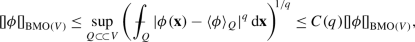(1.4)

for all $$\phi \in \operatorname{BMO}(V)$$, where the supremum is taken over all cubes $$Q$$ that are compactly supported in $$V$$ and have faces that are parallel to the coordinate planes. (If $$q=1$$ the quantity in the center of inequality (1.4) is equal to the $$\operatorname{BMO}$$-seminorm of $$\phi$$.) In Sect. 3 we then make use of (1.4) together with a version of Korn’s inequality due to Diening, Růžička, and Schumacher  to establish Korn’s inequality in $$\operatorname{BMO}$$, that is, (1.2).

In Sect. 4 we introduce our hypotheses on a compressible, nonlinearly hyperelastic body where the stored-energy density $$\sigma$$ depends on the material point $${\mathbf{x}}$$ and the right Cauchy-Green strain tensor $${\mathbf{C}}_{{\mathbf{u}}}({\mathbf{x}})=[\nabla {\mathbf{u}}({ \mathbf{x}})]^{\mathrm{T}}\nabla {\mathbf{u}}({\mathbf{x}})$$. Thus, in the absence of body forces and surface tractions, the total energy of a deformation $${\mathbf{u}}:\overline{\varOmega }\to {\mathbb{R}}^{n}$$, which satisfies $${\mathbf{u}}={\mathbf{d}}$$ on $${\mathscr {D}}\subset \partial {\varOmega }$$, is given by

$${\mathscr {E}}({\mathbf{u}})=\int _{\varOmega }\sigma \big({\mathbf{x}},{ \mathbf{C}}_{\mathbf{u}}({\mathbf{x}})\big)\;\!{\mathrm{d}}{\mathbf{x}}.$$

The second variation of ℰ evaluated at a solution of the corresponding equilibrium equations $${{\mathbf{u}}_{\mathrm{e}}}$$ is then equal to

\begin{aligned} \delta ^{2}{\mathscr {E}}({{\mathbf{u}}_{\mathrm{e}}})&[{\mathbf{w}},{ \mathbf{w}}] = \int _{\varOmega }{\mathbf{K}}\big({\mathbf{x}},{\mathbf{C}}_{{ \mathbf{u}}_{\mathrm{e}}}({\mathbf{x}})\big): \big[(\nabla {\mathbf{w}})^{ \mathrm{T}}\nabla {\mathbf{w}}\big]{\mathrm{d}}{\mathbf{x}} \\ &+\frac{1}{4}\int _{\varOmega }{\mathbf{E}}({\mathbf{x}}): {\mathbb{C}}\big({ \mathbf{x}},{\mathbf{C}}_{{\mathbf{u}}_{\mathrm{e}}}({\mathbf{x}})\big) \big[{\mathbf{E}}({\mathbf{x}})\big]{\mathrm{d}}{\mathbf{x}}, \end{aligned}

where $${\mathbb{C}}=4\frac{\partial ^{2}}{\partial {\mathbf{C}}^{2}}\sigma ({ \mathbf{x}},{\mathbf{C}})$$ denotes the elasticity tensor, $${\mathbf{K}}=2\frac{\partial }{\partial {\mathbf{C}}}\sigma ({\mathbf{x}},{ \mathbf{C}})$$ denotes the (second) Piola-Kirchhoff stress tensor, $${\mathbf{E}}=(\nabla {{\mathbf{u}}_{\mathrm{e}}})^{\mathrm{T}}\nabla { \mathbf{w}}+(\nabla {\mathbf{w}})^{\mathrm{T}}\nabla {{\mathbf{u}}_{ \mathrm{e}}}$$, and $${\mathbf{w}}\in W^{1,2}({\varOmega };{\mathbb{R}}^{n})$$ satisfies $${\mathbf{w}}=\mathbf{0}$$ on $${\mathscr {D}}\subset \partial {\varOmega }$$.

If $${\mathbf{K}}$$ is positive semi-definite, equivalently, the principal stresses are nonnegative, and ℂ is uniformly positive definite, then $$\delta ^{2}{\mathscr {E}}({{\mathbf{u}}_{\mathrm{e}}})$$ is uniformly positive. Standard techniques (see the introduction to  with particular attention to equations (1.3) and (1.4) on p. 411), which are usually applied in the space of deformation gradients, make use of Taylor’s theorem to deduce that there is then an $$L^{\infty }$$ neighborhood of $${\mathbf{C}}_{{\mathbf{u}}_{\mathrm{e}}}$$ in strain space (see (1.1)) in which there are no other solutions of the equilibrium equations. A refinement of this argument, which is due to John [30, pp. 624–625] (again, see the introduction to  with particular attention to equation (1.5) on p. 412), makes use of (1.3) to enlarge the set in which there are no other solutions to a neighborhood of $${\mathbf{C}}_{{\mathbf{u}}_{\mathrm{e}}}$$ in the space $$\operatorname{BMO}$$. We present the details of this argument in Sect. 5 of this manuscript. We also note, in Sect. 5.1, how these results simplify when one of the two right Cauchy-Green strain tensors is in an $$L^{\infty }$$-neighborhood of the reference configuration. Finally, in Sect. 6, we present further simplifications that occur when the reference configuration is itself at equilibrium.

## Preliminaries

For any domain (nonempty, connected, open set) $$U\subset {\mathbb{R}}^{n}$$, $$n\ge 2$$, we denote by $$L^{p}(U)$$, $$p\in [1,\infty )$$, the space of real-valued Lebesgue measurable functions $$\psi$$ whose $$L^{p}$$-norm is finite:

$$||\psi ||^{p}_{p,U} := \int _{U} |\psi ({\mathbf{x}})|^{p}\,{\mathrm{d}}{ \mathbf{x}}< \infty .$$

$$L^{\infty }(U)$$ will denote those Lebesgue measurable functions whose essential supremum is finite. $$L^{1}_{\operatorname{loc}}(U)$$ will consist of those Lebesgue measurable functions that are integrable on every compact subset of $$U$$. We shall write $$C(U;{\mathbb{R}}^{n})$$ for the set of continuous functions $${\mathbf{u}}:U\to {\mathbb{R}}^{n}$$, while $$C^{1}(\overline{U};{\mathbb{R}}^{n})$$ will denote those continuous functions $${\mathbf{u}}:\overline{U}\to {\mathbb{R}}^{n}$$ whose classical derivative exists on $$U$$ and has an extension that is continuous on $$\overline{U}$$, where $$\overline{U}$$ denotes the closure of $$U$$.

We shall write $${\varOmega }\subset {\mathbb{R}}^{n}$$, $$n\ge 2$$, to denote a Lipschitz domain, that is, a bounded domain whose boundary $$\partial {\varOmega }$$ is (strongly) Lipschitz. (See, e.g., [14, p. 127], [39, p. 72], or [26, Definition 2.5].) Essentially, a bounded domain is Lipschitz if, in a neighborhood of every boundary point, the boundary is the graph of a Lipschitz-continuous function and the domain is on “one side” of this graph.

For $$1\le p\le \infty$$, $$W^{1,p}(\varOmega ;{\mathbb{R}}^{N})$$ will denote the usual Sobolev space of (Lebesgue) measurable (vector-valued) functions $${\mathbf{u}}\in L^{p}(\varOmega ;{\mathbb{R}}^{N})$$ whose distributional gradient $$\nabla {\mathbf{u}}$$ is also contained in $$L^{p}$$. If $$\phi \in W^{1,p}(\varOmega ):=W^{1,p}(\varOmega ;{\mathbb{R}})$$ we shall denote its $$W^{1,p}$$-norm byFootnote 1

\begin{aligned} ||\phi ||_{W^{1,p}(\varOmega )} &:= \Big(||\phi ||^{p}_{p,\varOmega } +|| \nabla \phi ||^{p}_{p,\varOmega }\Big)^{\!1/p}, \quad 1\le p < \infty , \\ ||\phi ||_{W^{1,\infty }(\varOmega )} & := \max \{||\phi ||_{\infty , \varOmega }, ||\nabla \phi ||_{\infty ,\varOmega }\}, \quad p =\infty . \end{aligned}

We shall write $$W^{1,p}_{0}(\varOmega ;{\mathbb{R}}^{N})$$ for the subspace of $${\mathbf{u}}\in W^{1,p}(\varOmega ;{\mathbb{R}}^{N})$$ that satisfy $${\mathbf{u}}=\mathbf{0}$$ on $$\partial \varOmega$$ (in the sense of trace). $$W_{\operatorname{loc}}^{1,p}(U;{\mathbb{R}}^{N})$$ will denote the set of $${\mathbf{u}}\in W^{1,p}(V;{\mathbb{R}}^{N})$$ for every domain $$V{\subset \!\subset }U$$, where we write $$V{\subset \!\subset }U$$ provided that $$V\subset K_{V} \subset U$$ for some compact set $$K_{V}$$.

We shall write $${\mathbb{M}}^{n\times n}$$ for the (vector) space of $$n$$ by $$n$$ matrices with real entries. Given an orthonormal basis $${\mathbf{e}}_{i}$$, $$i=1,2,\dots ,n$$, for $${\mathbb{R}}^{n}$$ we write $$a_{i}={\mathbf{a}}\cdot {\mathbf{e}}_{i}$$ for $${\mathbf{a}}\in {\mathbb{R}}^{n}$$ and $$F_{ij}={\mathbf{e}}_{i}\cdot {\mathbf{F}}{\mathbf{e}}_{j}$$ for $${\mathbf{F}}\in {\mathbb{M}}^{n\times n}$$. The set of symmetric and positive-definite symmetric matrices in $${\mathbb{M}}^{n\times n}$$ shall be denoted by

\begin{aligned} \operatorname{Sym_{\mathit{n}}}&:=\{{\mathbf{E}}\in {\mathbb{M}}^{n\times n}: {\mathbf{E}}^{ \mathrm{T}}={\mathbf{E}}\}, \\ \operatorname{Psym_{\mathit{n}}}&:=\{{\mathbf{E}}\in \operatorname{Sym_{\mathit{n}}}: {\mathbf{a}}\cdot {\mathbf{E}}{ \mathbf{a}}>0 \text{ for all } {\mathbf{a}}\in {\mathbb{R}}^{n} \text{ with } {\mathbf{a}}\ne \mathbf{0}\}, \end{aligned}

respectively, where $${\mathbf{H}}^{\mathrm{T}}$$ denotes the transpose of $${\mathbf{H}}\in {\mathbb{M}}^{n\times n}$$. We write $${\mathbf{H}}:{\mathbf{K}}:=\operatorname{trace}({\mathbf{H}}{\mathbf{K}}^{\mathrm{T}})$$ for the inner product of $${\mathbf{H}},{\mathbf{K}}\in {\mathbb{M}}^{n\times n}$$. The norm of $${\mathbf{H}}\in {\mathbb{M}}^{n\times n}$$ is then given by $$|{\mathbf{H}}|:=\sqrt{{\mathbf{H}}:{\mathbf{H}}\,}$$. We write

$$\operatorname{SO}(n):=\{{\mathbf{Q}}\in {\mathbb{M}}^{n\times n}: {\mathbf{Q}}^{ \mathrm{T}}{\mathbf{Q}}={\mathbf{Q}}{\mathbf{Q}}^{\mathrm{T}}={\mathbf{I}}, \ \det {\mathbf{Q}}=1\}$$

for the group of rotations, where $${\mathbf{I}}$$ denotes the identity matrix and $$\det {\mathbf{F}}$$ denotes the determinant of $${\mathbf{F}}\in {\mathbb{M}}^{n\times n}$$.

### Strains and Geometric Rigidity

Fix $$p\ge 1$$. Given a mapping $${\mathbf{u}}\in W^{1,p}({\varOmega };{\mathbb{R}}^{n})$$ we define the right Cauchy-Green strain tensor $${\mathbf{C}}_{\mathbf{u}}\in L^{p/2}({\varOmega };\operatorname{Sym_{\mathit{n}}})$$ corresponding to $${\mathbf{u}}$$ by

$${\mathbf{C}}_{{\mathbf{u}}}:= (\nabla {\mathbf{u}})^{\mathrm{T}}\nabla { \mathbf{u}}.$$
(2.1)

This tensor can be used to measure the change in the length of the image of a curve in $${\varOmega }$$ after it is deformed by $${\mathbf{u}}$$ (see, e.g., [7, §1.8] or [25, §7.2]).

In  Friesecke, James, and Müller (see, also, Conti and Schweizer ) establish a Geometric-Rigidity result that implies that the distance (in $$L^{1}$$) from $${\mathbf{C}}_{{\mathbf{u}}}$$ to the identity matrix yields, up to a multiplicative constant, an upper bound for the distance (in $$L^{2}$$) from $$\nabla {\mathbf{u}}$$ to some particular rotation $${\mathbf{Q}}_{\mathbf{u}}\in \operatorname{SO}(n)$$. We shall make use of two interesting consequences of this result. The first is a theorem of Lorent [37, Theorem 1] that establishes conditions under which two mappings with the same strain tensor are related by a rigid deformation:

### Proposition 2.1

Let $${\mathbf{v}}\in W^{1,1}({\varOmega };{\mathbb{R}}^{n})$$ satisfy $$\det \nabla {\mathbf{v}}>0~a.e$$. Suppose that $${\mathbf{u}}\in W^{1,n}({\varOmega };{\mathbb{R}}^{n})$$ satisfies $$\det \nabla {\mathbf{u}}>0~a.e$$., $${\mathbf{C}}_{\mathbf{u}}={\mathbf{C}}_{\mathbf{v}}~a.e$$., and

$$|\nabla {\mathbf{u}}({\mathbf{x}})|^{n}\le K({\mathbf{x}}) \det \nabla { \mathbf{u}}({\mathbf{x}})\ \textit{for}\ a.e.~{\mathbf{x}}\in {\varOmega },$$

whereFootnote 2$$K\in L^{n}({\varOmega })$$. Then there exists a rotation $${\mathbf{R}}\in \operatorname{SO}(n)$$ such that

$$\nabla {\mathbf{v}}({\mathbf{x}}) ={\mathbf{R}}\nabla {\mathbf{u}}({ \mathbf{x}})\ \textit{ for }\ a.e.~{\mathbf{x}}\in {\varOmega }.$$

Ciarlet and Mardare have established a number of results that bound the distance between two mappings in a Sobolev space by a function of the distance between their right Cauchy-Green strain tensors in a corresponding Lebesgue space. The particular result we shall employ is [10, Theorem 3]:

### Proposition 2.2

Fix $$p\in (1,\infty )$$ and $$q\in [r,p]$$, where $$r:=\max \{1,p/2\}$$. Let $${\mathbf{v}}\in C^{1}({\overline{\varOmega }};{\mathbb{R}}^{n})$$ satisfy $$\det \nabla {\mathbf{v}}>0$$ in $${\overline{\varOmega }}$$. Suppose that $${\mathscr {D}}\subset \partial {\varOmega }$$ is nonempty and relatively open. Then there exists a constant $$C_{M}=C_{M}(p,q,{\mathbf{v}},{\varOmega },{\mathscr {D}})>0$$ such that

$$\int _{\varOmega }\big|{\mathbf{C}}_{\mathbf{u}}-{\mathbf{C}}_{\mathbf{v}} \big|^{q}\,{\mathrm{d}}{\mathbf{x}}\ge C_{M}\Big(\|{\mathbf{u}}-{ \mathbf{v}}\|_{W^{1,p}(\varOmega )}\Big)^{p}$$

for all $${\mathbf{u}}\in W^{1,2q}(\varOmega ;{\mathbb{R}}^{n})$$ that satisfy $$\det \nabla {\mathbf{u}}>0$$ $$a.e.$$ in $${\varOmega }$$ and $${\mathbf{u}}={\mathbf{v}}$$ on $${\mathscr {D}}$$.

### Remark 2.3

We note that $${\mathbf{C}}_{{\mathbf{u}}}={\mathbf{C}}_{{\mathbf{v}}}$$ does not necessarily imply that $${\mathbf{u}}={\mathbf{v}}$$ without further assumptions. See, e.g., Ciarlet and Mardare [9, p. 425], who attribute their counterexample to H. Le Dret (and a referee), or Lorent [37, p. 659].

### Bounded Mean Oscillation

We define the $$\operatorname{BMO}$$-seminormFootnote 3 of $$\psi \in L^{1}_{\operatorname{loc}}(U)$$ by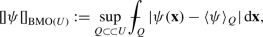(2.2)

where the supremum is to be taken over all nonempty, bounded (open) $$n$$-dimensional hypercubesFootnote 4$$Q$$ with faces parallel to the coordinate hyperplanes. Here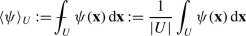denotes the average value of $$\psi$$ and $$|U|$$ denotes the $$n$$-dimensional Lebesgue measure of any bounded domain $$U\subset {\mathbb{R}}^{n}$$. The space $$\operatorname{BMO}(U)$$ (Bounded Mean Oscillation) is defined by

$$\operatorname{BMO}(U) := \{\psi \in L_{\operatorname{loc}}^{1}(U): {[\kern -0.3ex] \psi [\kern -0.3ex]}_{\operatorname{BMO}(U)} < \infty \}.$$
(2.3)

Note that one consequence of (2.2)–(2.3) is that $$L^{\infty }(U)\subset \operatorname{BMO}(U)$$ with

$${[\kern -0.3ex] \psi [\kern -0.3ex]}_{\operatorname{BMO}(U)} \le 2\|\psi \|_{\infty ,U}\ \text{ for all \psi \in L^{\infty }(U).}$$
(2.4)

We note for future reference that if $$U={\varOmega }$$, a Lipschitz domain, then a result of P. Jones  implies, in particular, that

$$\operatorname{BMO}({\varOmega })\subset L^{1}({\varOmega }).$$

It follows that

$$\|\psi \|_{\operatorname{BMO}({\varOmega })}={[\kern -0.3ex] \psi [\kern -0.3ex]}_{\operatorname{BMO}({\varOmega })} + | \langle \psi \rangle _{{\varOmega }}|$$
(2.5)

is a norm on $$\operatorname{BMO}({\varOmega })$$.

### Remark 2.4

1. The standard example of a function $$\phi \in \operatorname{BMO}({\mathbb{R}}^{n})$$ that is not bounded is $$\phi ({\mathbf{x}})=\ln |{\mathbf{x}}|$$. 2. There are a number of other equivalent seminorms on $$\operatorname{BMO}$$. The most ubiquitous involves the replacement of cubes $$Q$$ in (2.2) by open balls $$B{\subset \!\subset }U$$. Another possibility is the use of balls that get smaller as they approach the boundary (see Brezis and Nirenberg  who attribute such results to P. W. Jones ), i.e., the requirement that there is a fixed $$k\in (0,1)$$ such that each ball, $$B=B_{r}({\mathbf{x}})\subset \subset U$$ of radius $$r>0$$ and centered at $${\mathbf{x}}$$, satisfies

$$r\le k\operatorname{distance}({\mathbf{x}},\partial U).$$

Another useful equivalent seminorm is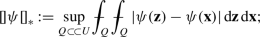in particular (see, e.g., [4, p. 6])

$${[\kern -0.3ex] \psi [\kern -0.3ex]}_{\operatorname{BMO}(U)}\le {[\kern -0.3ex] \psi [\kern -0.3ex]}_{*}\le 2{[\kern -0.3ex] \psi [\kern -0.3ex]}_{\operatorname{BMO}(U)}.$$

The monotone convergence theorem can then be used show that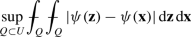is also an equivalent seminorm on $$\operatorname{BMO}(U)$$; it then follows that the seminorm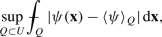which is used in [13, 43], is also equivalent to (2.2).

### Further Properties of BMO

One of the main properties of $$\operatorname{BMO}$$ that we shall use is contained in the following result. Although the proof can be found in , the significant analysis it is based upon is due to Fefferman and Stein , Iwaniec , and Diening, Růžička, and Schumacher .

### Proposition 2.5

Let $${\varOmega }\subset {\mathbb{R}}^{n}$$ be a LipschitzFootnote 5domain. Then, for all $$q\in [1,\infty )$$,

$$\operatorname{BMO}({\varOmega })\subset L^{q}({\varOmega })$$

with continuous injection, i.e., there is a constant $$J_{1}=J_{1}(q,{\varOmega })>0$$ such that, for every $$\psi \in \operatorname{BMO}({\varOmega })$$,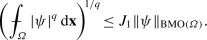(2.6)

Moreover, if $$1\le p< q<\infty$$, then there exists a constant $$J_{2}=J_{2}(p,q,{\varOmega })>0$$ such that every $$\psi \in \operatorname{BMO}({\varOmega })$$ satisfies

$$||\psi ||_{q,{\varOmega }} \le J_{2}\Big(||\psi ||_{\operatorname{BMO}({\varOmega })} \Big)^{1-p/q} \Big(||\psi ||_{p,{\varOmega }}\Big)^{p/q}.$$
(2.7)

In addition, the constants $$J_{i}$$ are scale invariant, that is, $$J_{i}(\lambda U +{\mathbf{a}})=J_{i}(U)$$ for every $$\lambda >0$$ and $${\mathbf{a}}\in {\mathbb{R}}^{n}$$. Here (see (2.2)) $$\|\cdot \|_{\operatorname{BMO}({\varOmega })}$$ is given by (2.5).

### Remark 2.6

Proposition 2.5 together with (2.4) shows that, for every $$p\in [1,\infty )$$,

$$L^{\infty }({\varOmega }) \subset \operatorname{BMO}({\varOmega })\subset L^{p}({\varOmega }).$$

Thus, $$\operatorname{BMO}$$ is a space that is “between” $$L^{\infty }$$ and all of the other $$L^{p}$$-spaces. However, researchers in Harmonic Analysis make use of $$\operatorname{BMO}$$ as a replacement for $$L^{\infty }$$. See, e.g., [45, §4.5].

The final result of this section follows from Proposition 2.5. However, since the result is a direct consequence of the scale invariance of the constant in the same result for cubes this result also follows from the original proof of John and Nirenberg .

### Corollary 2.7

Fix $$n\ge 2$$. Then, for every $$q\in (1,\infty )$$, there exists a constant $${\mathscr {N}}={\mathscr {N}}(n,q)$$ such that, for every bounded domain $$V\subset {\mathbb{R}}^{n}$$,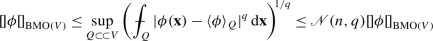(2.8)

for all $$\phi \in \operatorname{BMO}(V)$$.

### Remark 2.8

Corollary 2.7 shows that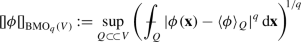is an equivalent seminorm on $$\operatorname{BMO}(V)$$. This result was first established by John and Nirenberg  when $$V=Q$$, a cube; it is there a consequence of what is now referred to as the John-Nirenberg inequality, that is, the exponential decay of the distribution function of $$|\phi -\langle \phi \rangle _{Q}|$$ for cubes. Inequality (2.8) is also well-known when $$V$$ and $$Q$$ are replaced by balls $$B{\subset \!\subset }\widehat{B}$$; see, e.g., Stein [45, pp. 144–146]. Stein also shows that, for balls $$\widehat{B}$$, the constant $${\mathscr {N}}$$ satisfies $${\mathscr {N}}(q,\widehat{B})\le q\widehat{{\mathscr {N}}}(\widehat{B})$$; the exponential decay of $$|\phi -\langle \phi \rangle _{\widehat{B}}|$$ for balls then follows from (2.8) and this growth estimate.

### Proof of Corollary 2.7

Let $$q\in (1,\infty )$$ and suppose that $$V\subset {\mathbb{R}}^{n}$$ is a bounded domain. Fix a cube $$Q {\subset \!\subset }V$$. Then, in view of (2.6) in Theorem 2.5 (with $${\varOmega }=Q$$) and (2.5), there exists a scale invariant constant $$J_{1}(q,Q)$$ such that, for all $$\psi \in \operatorname{BMO}({Q})$$,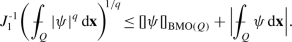(2.9)

Now, suppose that $$\phi \in \operatorname{BMO}(V)$$; then $$\phi \in \operatorname{BMO}(Q)$$. Define $$\psi :=\phi -\langle \phi \rangle _{Q }$$. Thus, $$\psi \in \operatorname{BMO}(Q)$$, $$\langle \psi \rangle _{Q }=0$$, and hence (2.9) yields(2.10)

Note that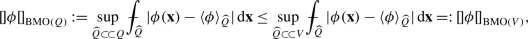which together with (2.10) and Hölder’s inequality yields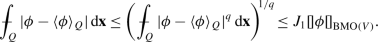(2.11)

The desired result, (2.8), now follows after taking the supremum of (2.11) over all cubes $$Q{\subset \!\subset }V$$ and noting that $${\mathscr {N}}(n,q):=J_{1}(n,q,Q)$$ is scale invariant and hence independent of the cube. □

## Korn’s Inequality

In this section we obtain a version of Korn’s inequality that involves the $$\operatorname{BMO}$$-seminorm of both the gradient of a function and the symmetric part of its gradient. Our result is a simple consequence of the following result of Diening, Růžička, and Schumacher.

### Proposition 3.1

([13, Theorem 5.17]) Let $${\varOmega }\subset {\mathbb{R}}^{n}$$, $$n\ge 2$$, be a bounded LipschitzFootnote 6domain. Suppose that $$q\in (1,\infty )$$. Then there exists a scale invariant constant $$K=K(q,{\varOmega })>0$$ such that, for all $${\mathbf{u}}\in W^{1,q}({\varOmega };{\mathbb{R}}^{n})$$,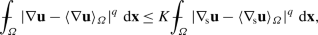(3.1)

where $${\nabla }_{\!\mathrm{s}}{\mathbf{u}}$$ denotes the symmetric part of the gradient of $${\mathbf{u}}$$, that is,

$${\nabla }_{\!\mathrm{s}}{\mathbf{u}}:= \frac{1}{2}\left [\nabla {\mathbf{u}}+( \nabla {\mathbf{u}})^{\mathrm{T}}\right ].$$

### Remark 3.2

The scale invariance of $$K$$ is clear since the average value of any function is scale invariant.

### Theorem 3.3

Fix $$n\ge 2$$. Then there exists a constant $${\mathscr {K}}={\mathscr {K}}(n)>0$$ such that, for any bounded domain $$U\subset {\mathbb{R}}^{n}$$,

$${[\kern -0.3ex] \nabla {\mathbf {u}}[\kern -0.3ex]}_{\operatorname{BMO}(U)}\le {\mathscr {K}}{[\kern -0.3ex] {\nabla }_{\!\mathrm {s}}{\mathbf {u}}[\kern -0.3ex]}_{ \operatorname{BMO}(U)},$$
(3.2)

for every $${\mathbf{u}}\in W_{\operatorname{loc}}^{1,1}(U;{\mathbb{R}}^{n})$$ with $$\nabla {\mathbf{u}}\in \operatorname{BMO}(U)$$.

### Remark 3.4

Note that the above inequality is valid for all bounded domains, unlike the standard Korn inequalities, which are valid only for John domains (see, e.g., ).

### Proof of Theorem 3.3

Fix a bounded domain $$U\subset {\mathbb{R}}^{n}$$. Let $${\mathbf{u}}\in W_{\operatorname{loc}}^{1,1}(U;{\mathbb{R}}^{n})$$ with $$\nabla {\mathbf{u}}\in \operatorname{BMO}(U)$$ and suppose that $$Q{\subset \!\subset }U$$ is a cube. The definition of $$\operatorname{BMO}(U)$$, (2.3), yields $$\nabla {\mathbf{u}}\in \operatorname{BMO}(Q)\cap L^{1}(Q)$$. Inequality (2.6) in Proposition 2.5 then implies that $$\nabla {\mathbf{u}}\in L^{q}(Q)$$ for every $$q\in [1,\infty )$$.

Next, by Korn’s inequality, Proposition 3.1, there exists a constant $$K=K(2,Q)$$, which is independent of $${\mathbf{u}}$$, such that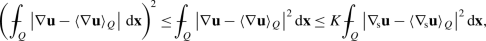(3.3)

where the first inequality in (3.3) follows from Hölder’s inequality. If we now take the supremum of (3.3) over all cubes $$Q{\subset \!\subset }U$$ and make use of the scale invariance of $$K$$ and the definition of the $$\operatorname{BMO}$$-seminorm, (2.2), we find that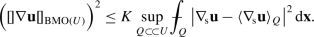(3.4)

The desired result, (3.2), now follows from (3.4), Corollary 2.7, and the fact that the constant $${\mathscr {N}}={\mathscr {N}}(n,q)$$ in (2.8) is independent of the domain. □

## Nonlinear Elasticity

In the remainder of this manuscript we shall focus on the minimization problem that arises when one considers the theory of Nonlinear (Finite) Hyperelasticity.

### The Constitutive Relation

We consider a body that for convenience we identify with the closure of a bounded Lipschitz domain $$\varOmega \subset {\mathbb{R}}^{n}$$, $$n=2$$ or $$n=3$$, which it occupies in a fixed reference configuration. A deformation of $$\overline{\varOmega }$$ is a mapping that lies in the space

$$\operatorname{Def}:=\{{\mathbf{u}}\in W^{1,2}(\varOmega ;{\mathbb{R}}^{n}): \det \nabla {\mathbf{u}}>0\ a.e.\},$$

where $$\det {\mathbf{F}}$$ denotes the determinant of $${\mathbf{F}}\in {\mathbb{M}}^{n\times n}$$. We assume that the body is composed of a hyperelastic material with stored-energy densityFootnote 7$$\sigma :\overline{\varOmega }\times \operatorname{Psym_{\mathit{n}}}\to [0,\infty )$$. The quantity $$\sigma ({\mathbf{x}},{\mathbf{C}}_{\mathbf{u}}({\mathbf{x}}))$$ gives the elastic energy stored at almost every point $${\mathbf{x}}\in \varOmega$$ of a deformation $${\mathbf{u}}\in \operatorname{Def}$$. Here, and in the sequel, $${\mathbf{C}}_{\mathbf{u}}=[\nabla {\mathbf{u}}]^{\mathrm{T}}\nabla { \mathbf{u}}$$, the right Cauchy-Green strain tensor (cf. (2.1)).

### Hypothesis 4.1

We assume that $$\sigma$$ satisfies the following:Footnote 8

(1):

$${\mathbf{C}}\mapsto \sigma ({\mathbf{x}},{\mathbf{C}}) \in C^{3}( \operatorname{Psym_{\mathit{n}}})$$, for $$a.e.~{\mathbf{x}}\in \varOmega$$;

(2):

$$({\mathbf{x}},{\mathbf{C}})\mapsto {{\mathrm{D}}}^{k} \sigma ({ \mathbf{x}},{\mathbf{C}})$$, $$k=0,1,2,3$$, are each (Lebesgue) measurable on their common domain $$\varOmega \times \operatorname{Psym_{\mathit{n}}}$$; and

(3):

$$({\mathbf{x}},{\mathbf{C}})\mapsto {{\mathrm{D}}}^{k} \sigma ({ \mathbf{x}},{\mathbf{C}})$$, $$k=0,1,2,3$$, are each bounded on $$\overline{\varOmega }\times \mathscr {K}$$ for every nonempty compact $$\mathscr {K}\subset \operatorname{Psym_{\mathit{n}}}$$. Here

$${{\mathrm{D}}}^{0} \sigma ({\mathbf{x}},{\mathbf{C}}):=\sigma ({ \mathbf{x}},{\mathbf{C}}), \qquad {{\mathrm{D}}}^{k} \sigma ({\mathbf{x}},{ \mathbf{C}}) := \frac{\partial ^{k}}{\partial {\mathbf{C}}^{k}}\sigma ({ \mathbf{x}},{\mathbf{C}})$$

denotes $$k$$-th derivative of the function $${\mathbf{C}}\mapsto \sigma (\cdot ,{\mathbf{C}})$$. We note, in particular, that

$${{\mathrm{D}}}\sigma :\overline{\varOmega }\times \operatorname{Psym_{\mathit{n}}}\to \operatorname{Sym_{\mathit{n}}}, \qquad {{\mathrm{D}}}^{2}\sigma :\overline{\varOmega }\times \operatorname{Psym_{\mathit{n}}}\to \operatorname{Lin}(\operatorname{Sym_{\mathit{n}}};\operatorname{Sym_{\mathit{n}}}),$$

where $$\operatorname{Lin}(\mathscr {U};\mathscr {V})$$ denotes the set of linear maps from the vector space $$\mathscr {U}$$ to the vector space $$\mathscr {V}$$. Thus, for every $${\mathbf{C}}\in \operatorname{Psym_{\mathit{n}}}$$, $${\mathbf{E}}\in \operatorname{Sym_{\mathit{n}}}$$, and almost every $${\mathbf{x}}\in {\varOmega }$$,

$${{\mathrm{D}}}\sigma ({\mathbf{x}},{\mathbf{C}})\in \operatorname{Sym_{\mathit{n}}}, \quad {{ \mathrm{D}}}^{2}\sigma ({\mathbf{x}},{\mathbf{C}})[{\mathbf{E}}]\in \operatorname{Sym_{\mathit{n}}}\!.$$

The second Piola-Kirchhoff stress tensor $${\mathbf{K}}$$ is defined to be twice the derivative of $$\sigma$$, i.e.,

$${\mathbf{K}}({\mathbf{x}},{\mathbf{C}}):= 2 \frac{\partial }{\partial {\mathbf{C}}}\sigma ({\mathbf{x}},{\mathbf{C}}) =2{{ \mathrm{D}}}\sigma ({\mathbf{x}},{\mathbf{C}}), \qquad {\mathbf{K}}: \overline{\varOmega }\times \operatorname{Psym_{\mathit{n}}}\to \operatorname{Sym_{\mathit{n}}},$$
(4.1)

while the first Piola-Kirchhoff stress tensor $${\mathbf{S}}$$ is given by

$${\mathbf{S}}({\mathbf{x}},{\mathbf{F}}) := {\mathbf{F}}{\mathbf{K}}({ \mathbf{x}},{\mathbf{F}}^{\mathrm{T}}{\mathbf{F}}), \qquad {\mathbf{S}}: \overline{\varOmega }\times {\mathbb{M}}_{+}^{n\times n}\to {\mathbb{M}}^{n \times n}\!,$$
(4.2)

where $${\mathbb{M}}_{+}^{n\times n}$$ denotes the set of $$n$$ by $$n$$ matrices with positive determinant. Although the tensor $${\mathbf{K}}$$ is the derivative of the stored energy, it is the tensor $${\mathbf{S}}$$ that is most convenient to use in the equilibrium (Euler-Lagrange) equations (see (4.10)–(4.11)). For any injective deformation $${\mathbf{u}}\in \operatorname{Def}\cap \, C^{1}({\overline{\varOmega }};{\mathbb{R}}^{n})$$, the Cauchy stress tensor $${\mathbf{T}}={\mathbf{T}}({\mathbf{y}})\in \operatorname{Sym_{\mathit{n}}}$$ is given by

$${\mathbf{T}}({\mathbf{y}}) :={\mathbf{F}}{\mathbf{K}}({\mathbf{x}},{ \mathbf{F}}^{\mathrm{T}}{\mathbf{F}}){\mathbf{F}}^{\mathrm{T}}(\det { \mathbf{F}})^{{\text{-}}1}, \quad \text{{\mathbf{F}}:=\nabla {\mathbf{u}}({\mathbf{x}}), {\mathbf{y}}={\mathbf{u}}({\mathbf{x}}).}$$
(4.3)

The eigenvalues of $${\mathbf{T}}({\mathbf{y}})$$ are called the principal stresses at $${\mathbf{y}}\in {\mathbf{u}}({\varOmega })$$. The elasticity tensor ℂ is defined to be four times the second derivative of $${\mathbf{C}}\mapsto \sigma ({\mathbf{x}},{\mathbf{C}})$$, that is,

$${\mathbb{C}}({\mathbf{x}},{\mathbf{C}}):=4 \frac{\partial ^{2}}{\partial {\mathbf{C}}^{2}} \sigma ({\mathbf{x}},{ \mathbf{C}}) =4{{\mathrm{D}}}^{2}\sigma ({\mathbf{x}},{\mathbf{C}}).$$
(4.4)

In view of the symmetry of the second gradient,

$${\mathbf{B}}:{\mathbb{C}}({\mathbf{x}},{\mathbf{C}})[{\mathbf{E}}]={ \mathbf{E}}:{\mathbb{C}}({\mathbf{x}},{\mathbf{C}})[{\mathbf{B}}]$$

for all $${\mathbf{C}}\in \operatorname{Psym_{\mathit{n}}}$$ and all $${\mathbf{B}},{\mathbf{E}}\in \operatorname{Sym_{\mathit{n}}}$$.

### Definition 4.2

The elasticity tensor is said to be uniformly positive definite at a deformation $${\mathbf{u}}\in \operatorname{Def}$$ provided that there exists a constant $$c>0$$ such that, for every $${\mathbf{E}}\in \operatorname{Sym_{\mathit{n}}}$$ and $$a.e.$$ $${\mathbf{x}}\in \varOmega$$,

$${\mathbf{E}}:{\mathbb{C}}\big({\mathbf{x}},{\mathbf{C}}_{\mathbf{u}}({ \mathbf{x}})\big)[{\mathbf{E}}]\ge c |{\mathbf{E}}|^{2},$$

where $${\mathbf{C}}_{\mathbf{u}}=(\nabla {\mathbf{u}})^{\mathrm{T}}\nabla { \mathbf{u}}$$. The reference configuration is said to be stress free provided that,

$${\mathbf{K}}({\mathbf{x}},{\mathbf{I}}) =\mathbf{0}\ \text{ for a.e.~{\mathbf{x}}\in \varOmega },$$

where $${\mathbf{I}}\in {\mathbb{M}}^{n\times n}$$ denotes the identity matrix.

### Remark 4.3

Let $$n=3$$ and suppose that $${\mathbf{u}}\in \operatorname{Def}\cap \, C^{1}(\varOmega ;{\mathbb{R}}^{n})$$ is injective. Further, let $$\varGamma \subset {\mathbf{u}}({\varOmega })$$ be a smooth, oriented surface with continuous outward unit normal field $${\mathbf{y}}\mapsto {\mathbf{m}}({\mathbf{y}})$$, $${\mathbf{y}}\in \varGamma$$. If $${\mathbf{x}}\mapsto {\mathbf{K}}({\mathbf{x}},{\mathbf{C}})$$ is continuous on $${\varOmega }$$, then, for any $${\mathbf{y}}={\mathbf{u}}({\mathbf{x}})$$ with $${\mathbf{y}}\in \varGamma$$,

$${\mathbf{T}}({\mathbf{y}}){\mathbf{m}}({\mathbf{y}}),$$

gives the force, per unit (deformed) area, exerted across $$\varGamma$$ upon the material on the negative side of $$\varGamma$$ by the material on the positive side $$\varGamma$$ (see, e.g., [24, p. 97] or [25, §19]).

### Remark 4.4

One can alternatively assume that the stored-energy function depends on the deformation gradient $$\nabla {\mathbf{u}}$$. In this case one postulates a (frame-indifferent) function $$W:\overline{\varOmega }\times {\mathbb{M}}_{+}^{n\times n}\to [0,\infty )$$, which will satisfy, for $$a.e.~{\mathbf{x}}\in {\varOmega }$$,

$$W({\mathbf{x}},{\mathbf{F}}) = \sigma ({\mathbf{x}},{\mathbf{F}}^{ \mathrm{T}}{\mathbf{F}})\ \text{ for every {\mathbf{F}}\in {\mathbb{M}}_{+}^{n\times n}}.$$
(4.5)

In this formulation one usually defines the elasticity tensor as the second derivative of $$W$$ with respect to $${\mathbf{F}}$$, that is,

$${\mathbb{A}}({\mathbf{x}},{\mathbf{F}}):= \frac{\partial ^{2}}{\partial {\mathbf{F}}^{2}} W({\mathbf{x}},{ \mathbf{F}}).$$
(4.6)

If we now twice differentiate (4.5) (see, e.g., [43, Lemma 5.4]), we conclude, with the aid of (4.1), (4.4), (4.6), and the symmetry of the second derivative, that

\begin{aligned} {\mathbf{H}}:{\mathbb{A}}({\mathbf{x}},{\mathbf{F}})[{\mathbf{H}}] &= \frac{1}{2}({\mathbf{H}}^{\mathrm{T}}{\mathbf{F}}+{\mathbf{F}}^{\mathrm{T}}{ \mathbf{H}}): {\mathbb{C}}({\mathbf{x}},{\mathbf{F}}^{\mathrm{T}}{ \mathbf{F}})\big[\frac{1}{2}({\mathbf{H}}^{\mathrm{T}}{\mathbf{F}}+{ \mathbf{F}}^{\mathrm{T}}{\mathbf{H}})\big] \\ &\quad +{\mathbf{K}}({\mathbf{x}},{\mathbf{F}}^{\mathrm{T}}{\mathbf{F}}):({ \mathbf{H}}^{\mathrm{T}}{\mathbf{H}}), \end{aligned}
(4.7)

for all $${\mathbf{F}}\in {\mathbb{M}}_{+}^{n\times n}$$ and $${\mathbf{H}}\in {\mathbb{M}}^{n\times n}$$. In particular, when the reference configuration is stress free, it follows that

$${\mathbf{H}}:{\mathbb{A}}({\mathbf{x}},{\mathbf{I}})[{\mathbf{H}}] = \frac{1}{2}({\mathbf{H}}^{\mathrm{T}}+ {\mathbf{H}}): {\mathbb{C}}({ \mathbf{x}},{\mathbf{I}})\big[\frac{1}{2}({\mathbf{H}}^{\mathrm{T}}+ { \mathbf{H}})\big].$$

Thus, both $${\mathbb{C}}({\mathbf{x}},{\mathbf{I}})$$ and $${\mathbb{A}}({\mathbf{x}},{\mathbf{I}})$$ correspond to the classical elasticity tensor used in the linear theory (see, e.g., ).

### Equilibrium Solutions and Energy Minimizers

$$\partial \varOmega = \overline{{\mathscr {D}}} \cup \overline{{\mathscr {S}}}\quad \text{with {\mathscr {D}} and {\mathscr {S}} relatively open and } { \mathscr {D}}\cap {\mathscr {S}}=\varnothing .$$

In addition, we shall suppose that $${\mathscr {D}}\ne \varnothing$$. We assume that a Lipschitz-continuous function $${\mathbf{d}}:{\mathscr {D}}\to {\mathbb{R}}^{n}$$ is prescribed; $${\mathbf{d}}$$ will give the deformation of $${\mathscr {D}}$$. If $${\mathscr {S}}\ne \varnothing$$ we assume that a function $${\mathbf{s}}\in L^{2}({\mathscr {S}};{\mathbb{R}}^{n})$$ is prescribed; for $${\mathscr {H}}^{n-1}$$-$$a.e.~{\mathbf{x}}\in {\mathscr {S}}$$, $${\mathbf{s}}({\mathbf{x}})$$ will give the surface force (per unit area when $$n=3$$) exerted on the body at the point $${\mathbf{x}}$$ by its environment. Here $${\mathscr {H}}^{k}$$ denotes $$k$$-dimensional Hausdorff measureFootnote 9 (see, e.g., [14, Chap. 2]). Finally, we suppose that a function $${\mathbf{b}}\in L^{2}(\varOmega ;{\mathbb{R}}^{n})$$ is prescribed; for $$a.e.~{\mathbf{x}}\in \varOmega$$, $${\mathbf{b}}({\mathbf{x}})$$ will give the body force (per unit volume when $$n=3$$) exerted on the body at the point $${\mathbf{x}}$$ by its environment. The set of Admissible Deformations will be denoted by

$$\operatorname{AD}:=\{{\mathbf{u}}\in \operatorname{Def}\cap \, W^{1,\infty }(\varOmega ;{ \mathbb{R}}^{n}): {\mathbf{u}}={\mathbf{d}}\text{ on {\mathscr {D}}}\}.$$

The total energy of an admissible deformation $${\mathbf{u}}\in \operatorname{AD}$$ is defined to be

$${\mathscr {E}}({\mathbf{u}}):=\int _{\varOmega }\Big[\sigma \big({\mathbf{x}},{ \mathbf{C}}_{\mathbf{u}}({\mathbf{x}})\big) -{\mathbf{b}}({\mathbf{x}}) \cdot {\mathbf{u}}({\mathbf{x}})\Big]{\mathrm{d}}{\mathbf{x}}-\int _{{ \mathscr {S}}} {\mathbf{s}}({\mathbf{x}})\cdot {\mathbf{u}}({\mathbf{x}})\,{ \mathrm{d}}{\mathscr {H}}^{n-1}_{\mathbf{x}}$$
(4.8)

with $${\mathbf{C}}_{\mathbf{u}}:=(\nabla {\mathbf{u}})^{\mathrm{T}}\nabla { \mathbf{u}}$$. The first variation of ℰ is given by

\begin{aligned} \delta {\mathscr {E}}({\mathbf{u}})[{\mathbf{w}}]=&\int _{\varOmega }{{ \mathrm{D}}}\sigma \big({\mathbf{x}},{\mathbf{C}}_{\mathbf{u}}({\mathbf{x}}) \big): \Big(\big[\nabla {\mathbf{u}}({\mathbf{x}})\big]^{\mathrm{T}} \nabla {\mathbf{w}}({\mathbf{x}}) +\big[\nabla {\mathbf{w}}({\mathbf{x}}) \big]^{\mathrm{T}}\nabla {\mathbf{u}}({\mathbf{x}})\Big){\mathrm{d}}{ \mathbf{x}} \\ &-\int _{\varOmega }{\mathbf{b}}({\mathbf{x}})\cdot {\mathbf{w}}({\mathbf{x}}) \,{\mathrm{d}}{\mathbf{x}}-\int _{{\mathscr {S}}} {\mathbf{s}}({\mathbf{x}}) \cdot {\mathbf{w}}({\mathbf{x}})\,{\mathrm{d}}{\mathscr {H}}^{n-1}_{ \mathbf{x}}, \end{aligned}

for all variations $${\mathbf{w}}\in \operatorname{Var}$$, where

$$\operatorname{Var}:=\{{\mathbf{w}}\in W^{1,2}(\varOmega ;{\mathbb{R}}^{n}): {\mathbf{w}}= \mathbf{0} \text{ on {\mathscr {D}}}\}.$$

The second variation of ℰ is then given by (see (4.1), (4.4), and (4.7))

\begin{aligned} \delta ^{2}{\mathscr {E}}({\mathbf{u}})&[{\mathbf{w}},{\mathbf{w}}] = \int _{\varOmega }{\mathbf{K}}\big({\mathbf{x}},{\mathbf{C}}_{\mathbf{u}}({ \mathbf{x}})\big): \big[(\nabla {\mathbf{w}})^{\mathrm{T}}\nabla { \mathbf{w}}\big]{\mathrm{d}}{\mathbf{x}} \\ &+\frac{1}{4}\int _{\varOmega }\big[(\nabla {\mathbf{u}})^{\mathrm{T}}\nabla { \mathbf{w}}+(\nabla {\mathbf{w}})^{\mathrm{T}}\nabla {\mathbf{u}}\big]: { \mathbb{C}}\big({\mathbf{x}},{\mathbf{C}}_{\mathbf{u}}({\mathbf{x}})\big) \big[(\nabla {\mathbf{u}})^{\mathrm{T}}\nabla {\mathbf{w}}+(\nabla { \mathbf{w}})^{\mathrm{T}}\nabla {\mathbf{u}}\big]\,{\mathrm{d}}{\mathbf{x}}. \end{aligned}
(4.9)

### Remark 4.5

It is clear from (4.9) that the positivity of the second variation, i.e., $$\delta ^{2}{\mathscr {E}}({\mathbf{u}})\ge 0$$, is not a consequence of the positivity of the elasticity tensor ℂ alone. However, the second variation is positive whenever both ℂ and $${\mathbf{K}}$$ are positive definite (see Lemma 4.6).

We shall assume that we are given a deformation $${\mathbf{u}}_{\mathrm{e}}\in \operatorname{AD}$$ that is a weak solution of the Equilibrium Equations corresponding to (4.8), i.e., $$\delta {\mathscr {E}}({\mathbf{u}})=0$$ or, equivalently,

$$0=\int _{\varOmega }\Big[{\mathbf{S}}\big({\mathbf{x}},\nabla {\mathbf{u}}_{ \mathrm{e}}({\mathbf{x}})\big) :\nabla {\mathbf{w}}({\mathbf{x}}) -{ \mathbf{b}}({\mathbf{x}})\cdot {\mathbf{w}}({\mathbf{x}})\Big]{\mathrm{d}}{ \mathbf{x}}-\int _{\mathscr {S}}{\mathbf{s}}({\mathbf{x}})\cdot {\mathbf{w}}({ \mathbf{x}})\,{\mathrm{d}}{\mathscr {H}}^{n-1}_{\mathbf{x}}$$
(4.10)

for all $${\mathbf{w}}\in \operatorname{Var}$$, where $${\mathbf{S}}$$ is given by (4.1)–(4.2). If $${\mathscr {D}}=\partial {\varOmega }$$ we shall call $${\mathbf{u}}_{\mathrm{e}}$$ a solution of the (pure) displacement problem. Otherwise, we shall refer to such a $${\mathbf{u}}_{\mathrm{e}}$$ as a solution of the (genuine) mixed problem. If in addition $$\sigma \in C^{2}({\overline{\varOmega }}\times \operatorname{Psym_{\mathit{n}}})$$ and $${\mathbf{u}}_{\mathrm{e}}\in C^{2}(\varOmega ;{\mathbb{R}}^{n})\cap C^{1}( \overline{\varOmega };{\mathbb{R}}^{n})$$, then $${\mathbf{u}}_{\mathrm{e}}$$ will be a classical solution of the equations of equilibrium (see, e.g., [7, §2.6], [24, §27], or [25, §49]), i.e., $${\mathbf{u}}_{\mathrm{e}}$$ will satisfy

$$\begin{gathered} \operatorname{Div}{\mathbf{S}}(\nabla {\mathbf{u}}_{\mathrm{e}}) +{\mathbf{b}}= \mathbf{0}\ \text{ in \varOmega ,} \\ {\mathbf{S}}(\nabla {\mathbf{u}}_{\mathrm{e}}){\mathbf{n}}= {\mathbf{s}}\ \text{ on {\mathscr {S}},}\qquad {\mathbf{u}}_{\mathrm{e}}={\mathbf{d}}\ \text{ on {\mathscr {D}},} \end{gathered}$$
(4.11)

where $${\mathbf{n}}({\mathbf{x}})$$ denotes the outward unit normal to $$\varOmega$$ at $${\mathscr {H}}^{n-1}$$-$$a.e.~{\mathbf{x}}\in {\mathscr {S}}$$ and $$\operatorname{Div}{\mathbf{M}}\in {\mathbb{R}}^{n}$$ is given by $$(\operatorname{Div}{\mathbf{M}})_{i}=\sum _{j} \frac{\partial }{\partial {\mathbf{x}}_{j}} M_{ij}$$.

We are interested in the local minimality (in an appropriate topology) of solutions of (4.10). For future use we note that, for every $${\mathbf{u}},{\mathbf{v}}\in \operatorname{AD}$$, (4.8) gives us

$${\mathscr {E}}({\mathbf{v}})-{\mathscr {E}}({\mathbf{u}}) = \int _{\varOmega }\big[\sigma \big({\mathbf{C}}_{\mathbf{v}}\big)-\sigma \big({\mathbf{C}}_{ \mathbf{u}}\big) -{\mathbf{b}}\cdot {\mathbf{w}}\big]{\mathrm{d}}{ \mathbf{x}}- \int _{\mathscr {S}}{\mathbf{s}}\cdot {\mathbf{w}}\,{ \mathrm{d}}{\mathscr {H}}^{n-1}_{\mathbf{x}},$$

where $${\mathbf{w}}:={\mathbf{v}}-{\mathbf{u}}\in W^{1,\infty }(\varOmega ;{ \mathbb{R}}^{N}) \cap \operatorname{Var}$$. It follows that, when $${\mathbf{u}}_{\mathrm{e}}\in \operatorname{AD}$$ is a solution of the equilibrium equations, (4.10), we have the identity, for every $${\mathbf{v}}\in \operatorname{AD}$$,

$${\mathscr {E}}({\mathbf{v}})-{\mathscr {E}}({\mathbf{u}}_{\mathrm{e}}) = \int _{\varOmega }\Big[\sigma \big({\mathbf{x}},{\mathbf{C}}_{\mathbf{v}}({ \mathbf{x}})\big)- \sigma \big({\mathbf{x}},{\mathbf{C}}_{\mathrm{e}}({ \mathbf{x}})\big) -{\mathbf{S}}\big({\mathbf{x}},\nabla {\mathbf{u}}_{ \mathrm{e}}({\mathbf{x}})\big) :\nabla {\mathbf{w}}({\mathbf{x}})\Big]{ \mathrm{d}}{\mathbf{x}},$$
(4.12)

where $${\mathbf{C}}_{\mathrm{e}}:={\mathbf{C}}_{{\mathbf{u}}_{\mathrm{e}}}=( \nabla {\mathbf{u}}_{\mathrm{e}})^{\mathrm{T}}\nabla {\mathbf{u}}_{ \mathrm{e}}$$, $${\mathbf{C}}_{\mathbf{v}}:=(\nabla {\mathbf{v}})^{\mathrm{T}}\nabla { \mathbf{v}}$$, and $${\mathbf{w}}:={\mathbf{v}}-{\mathbf{u}}$$.

### Multiaxial Tension

In the sequel we shall assume that the second Piola-Kirchhoff stress tensor $${\mathbf{K}}$$ is positive semidefinite at a given deformation $${\mathbf{v}}\in \operatorname{AD}$$, that is, for $$a.e.~{\mathbf{x}}\in {\varOmega }$$,

$${\mathbf{a}}\cdot {\mathbf{K}}\big({\mathbf{x}},{\mathbf{C}}_{\mathbf{v}}({ \mathbf{x}})\big){\mathbf{a}}\ge 0\ \text{ for every {\mathbf{a}}\in {\mathbb{R}}^{n}.}$$
(4.13)

In view of (4.3) and the positivity of the Jacobian $$\det \nabla {\mathbf{v}}$$, inequality (4.13) is essentially the same as the assumption that the Cauchy stress tensor $${\mathbf{T}}$$ is positive semidefinite. Thus, (4.13) is the assumption that the principal stresses in the deformed material are all tensile.

The next result yields a simple consequence of (4.13) that we shall use. We sketch a proof for the convenience of the reader.

### Lemma 4.6

Let $${\mathbf{L}}\in L^{p}({\varOmega };\operatorname{Sym_{\mathit{n}}})$$ for some $$p\in [1,\infty ]$$. Suppose that, at almost every $${\mathbf{x}}\in {\varOmega }$$, $${\mathbf{L}}({\mathbf{x}})$$ is positive semidefinite. Then

$$I({\mathbf{w}})=\int _{\varOmega }\big(\big[\nabla {\mathbf{w}}({\mathbf{x}}) \big]^{\mathrm{T}}\nabla {\mathbf{w}}({\mathbf{x}})\big):{\mathbf{L}}({ \mathbf{x}}) \,{\mathrm{d}}{\mathbf{x}}\ge 0$$
(4.14)

for all $${\mathbf{w}}\in W^{1,q}({\varOmega };{\mathbb{R}}^{n})$$, where

$$\frac{1}{p}+\frac{2}{q}=1.$$
(4.15)

Conversely, suppose that $${\mathbf{L}}\in C({\varOmega };\operatorname{Sym_{\mathit{n}}})$$ satisfies (4.14) for all $${\mathbf{w}}\in W_{0}^{1,2}({\varOmega };{\mathbb{R}}^{n})$$. Then $${\mathbf{L}}({\mathbf{x}})$$ is positive semidefinite at every $${\mathbf{x}}\in {\varOmega }$$.

### Proof

Fix $$p\in [1,\infty ]$$. Let $${\mathbf{L}}\in L^{p}({\varOmega };\operatorname{Sym_{\mathit{n}}})$$ with $${\mathbf{L}}({\mathbf{x}})$$ positive semidefinite at $$a.e.~{\mathbf{x}}\in {\varOmega }$$. Then, by the spectral theorem, at $$a.e.~{\mathbf{x}}\in {\varOmega }$$ there exists an orthonormal basis $${\mathbf{e}}_{k}({\mathbf{x}})$$ and scalars $$\alpha _{k}({\mathbf{x}})$$, $$k=1,2,\ldots ,n$$, with $$\alpha _{k}\ge 0~a.e$$. (and $${\mathbf{x}}\mapsto \alpha _{k}({\mathbf{x}})$$ and $${\mathbf{x}}\mapsto {\mathbf{e}}_{k}({\mathbf{x}})$$ measurable), such that

$${\mathbf{L}}({\mathbf{x}})=\sum _{k=1}^{n} \alpha _{k}({\mathbf{x}}){ \mathbf{e}}_{k}({\mathbf{x}})\otimes {\mathbf{e}}_{k}({\mathbf{x}}),$$
(4.16)

where $${\mathbf{a}}\otimes {\mathbf{b}}\in {\mathbb{M}}^{n\times n}$$ is defined by $$[{\mathbf{a}}\otimes {\mathbf{b}}]{\mathbf{c}}=({\mathbf{b}}\cdot { \mathbf{c}}){\mathbf{a}}$$ for every $${\mathbf{c}}\in {\mathbb{R}}^{n}$$.

Let $${\mathbf{w}}\in W^{1,q}({\varOmega };{\mathbb{R}}^{n})$$, where $$q$$ satisfies (4.15). Then (4.16) yields, with the aid of the inequalities $$\alpha _{k}\ge 0~a.e$$.,

$$\big(\big[\nabla {\mathbf{w}}\big]^{\mathrm{T}}\nabla {\mathbf{w}}\big):{ \mathbf{L}}= \sum _{k=1}^{n} \alpha _{k}\big|(\nabla {\mathbf{w}}){ \mathbf{e}}_{k}\big|^{2}\ge 0~a.e.$$
(4.17)

Since $$[\nabla {\mathbf{w}}]^{\mathrm{T}}\nabla {\mathbf{w}}\in L^{q/2}({ \varOmega };\operatorname{Sym_{\mathit{n}}})$$ and $${\mathbf{L}}\in L^{p}({\varOmega };\operatorname{Sym_{\mathit{n}}})$$, equation (4.15) implies that (4.17) is integrable. Thus, we may integrate (4.17) over $${\varOmega }$$ to arrive at (4.14).

Conversely, suppose that $${\mathbf{L}}\in C({\varOmega };\operatorname{Sym_{\mathit{n}}})$$ satisfies (4.14) for all $${\mathbf{w}}\in W_{0}^{1,2}({\varOmega };{\mathbb{R}}^{n})$$. Note that (4.14) is the condition that $$I$$ assumes its infimum at $${\mathbf{w}}=\mathbf{0}$$. A standard result (see, e.g.,  or [2, Theorem 2.2(i)]) is that $$I$$ is then quasiconvex at $${\mathbf{w}}=\mathbf{0}$$; thus, for every $${\mathbf{x}}_{\mathrm{o}}\in {\varOmega }$$ and $$\widehat{{\mathbf{w}}}\in W_{0}^{1,2}(B;{\mathbb{R}}^{n})$$,

$$\widehat{I} (\widehat{{\mathbf{w}}})= \int _{B} \big(\big[\nabla \widehat{{\mathbf{w}}}({\mathbf{z}})\big]^{\mathrm{T}}\nabla \widehat{{\mathbf{w}}}({\mathbf{z}})\big):{\mathbf{L}}({\mathbf{x}}_{ \mathrm{o}}) \,{\mathrm{d}}{\mathbf{z}}\ge 0,$$
(4.18)

where $$B\subset {\mathbb{R}}^{n}$$ denotes the unit ball centered at $$\mathbf{0}$$. In particular, fix $${\mathbf{e}}\in {\mathbb{R}}^{n}$$ with $$|{\mathbf{e}}|=1$$ and let $$\widehat{{\mathbf{w}}}=\phi \;\!{\mathbf{e}}$$, where $$\phi \in W_{0}^{1,2}(B)$$. We then find, with the aid of the spectral theorem (see (4.16)), that (4.18) reduces to

$$0\le \widehat{I} (\phi \;\!{\mathbf{e}})= \int _{B} \nabla \phi \cdot { \mathbf{L}}({\mathbf{x}}_{\mathrm{o}})\nabla \phi \,{\mathrm{d}}{ \mathbf{z}}= \sum _{k=1}^{n} \alpha _{k}\int _{B}|\nabla \phi \cdot { \mathbf{e}}_{k}|^{2}{\mathrm{d}}{\mathbf{z}},$$

for all $$\phi \in W_{0}^{1,2}(B)$$. The nonnegativity of the constant eigenvalues $$\alpha _{k}$$, which yields $${\mathbf{L}}({\mathbf{x}}_{\mathrm{o}})$$ positive semidefinite, now follows from an appropriate choice of $$\phi$$ (see, e.g., Truesdell and Noll [47, §68bis] or Dacorogna [12, p. 84]). □

### The Elasticity Tensor

If the elasticity tensor is uniformly positive definite at a deformation $${\mathbf{u}}\in \operatorname{Def}\cap \, W^{1,\infty }({\varOmega };{\mathbb{R}}^{n})$$, i.e.,

$${\mathbf{M}}:{\mathbb{C}}\big({\mathbf{x}},{\mathbf{C}}_{\mathbf{u}}({ \mathbf{x}})\big)[{\mathbf{M}}]\ge 2\beta |{\mathbf{M}}|^{2},$$
(4.19)

for some $$\beta >0$$, every $${\mathbf{M}}\in \operatorname{Sym_{\mathit{n}}}$$, and $$a.e.~{\mathbf{x}}\in \varOmega$$, then the choice $${\mathbf{M}}={\mathbf{B}}({\mathbf{x}})$$ together with an integration of (4.19) yields

$$\int _{\varOmega }{\mathbf{B}}({\mathbf{x}}):{\mathbb{C}}\big({\mathbf{x}},{ \mathbf{C}}_{\mathbf{u}}({\mathbf{x}})\big) \big[{\mathbf{B}}({\mathbf{x}}) \big]\,{\mathrm{d}}{\mathbf{x}}\ge 2\beta \int _{\varOmega }|{\mathbf{B}}({ \mathbf{x}})|^{2}{\mathrm{d}}{\mathbf{x}}.$$
(4.20)

### Lemma 4.7

Let $$\sigma$$ satisfy (1)(3) of Hypothesis 4.1. Suppose that $${\mathbf{u}}\in \operatorname{AD}$$ satisfies (4.20), for some $$\beta >0$$ and all $${\mathbf{B}}\in L^{2}({\varOmega };\operatorname{Sym_{\mathit{n}}})$$. Moreover, assume that

$${\mathbf{C}}_{\mathbf{u}}({\mathbf{x}})\in {\mathscr {B}}\ \textit{for}\ a.e.~{\mathbf{x}}\in \varOmega ,$$
(4.21)

whereis a nonempty, bounded, open set with $$\overline{{\mathscr {B}}}\subset \operatorname{Psym_{\mathit{n}}}$$ and $${\mathbf{C}}_{\mathbf{u}}:=(\nabla {\mathbf{u}})^{\mathrm{T}}\nabla { \mathbf{u}}$$. Then there exists an $$\varepsilon >0$$ such that any $${\mathbf{v}}\in \operatorname{AD}$$ that satisfies, for $$a.e.~{\mathbf{x}}\in \varOmega$$,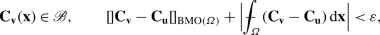(4.22)

$${\mathbf{C}}_{\mathbf{v}}:=(\nabla {\mathbf{v}})^{\mathrm{T}}\nabla { \mathbf{v}}$$, will also satisfy

$$\int _{\varOmega }{\mathbf{E}}({\mathbf{x}}):{\mathbb{C}}\big({\mathbf{x}},{ \mathbf{C}}_{\mathbf{v}}({\mathbf{x}})\big) \big[{\mathbf{E}}({\mathbf{x}}) \big]\,{\mathrm{d}}{\mathbf{x}}\ge \beta \int _{\varOmega }\big|{\mathbf{E}}({ \mathbf{x}})\big|^{2}\,{\mathrm{d}}{\mathbf{x}}, \quad {\mathbf{E}}:={ \mathbf{C}}_{\mathbf{v}}-{\mathbf{C}}_{\mathbf{u}}.$$
(4.23)

Here and in the sequel, we use the notation $${[\kern -0.3ex] {\mathbf {C}}_{{\mathbf {v}}} -{\mathbf {C}}_{{\mathbf {u}}} [\kern -0.3ex]}_{\operatorname{BMO}(\varOmega )}$$ to denote the $$\operatorname{BMO}$$-seminorm of the tensor $${\mathbf{C}}_{\mathbf{v}}-{\mathbf{C}}_{\mathbf{u}}$$. The definition is precisely as in (2.2), except one has the tensor in place of $$\psi$$ and the Euclidean norm in place of the absolute value in the integral.

### Proof of Lemma 4.7

For clarity of exposition, we suppress the variable $${\mathbf{x}}$$. Let $${\mathbf{u}}\in \operatorname{AD}$$ satisfy (4.20) and (4.21) for all $${\mathbf{B}}\in L^{2}({\varOmega };\operatorname{Sym_{\mathit{n}}})$$. Suppose that $${\mathbf{v}}\in \operatorname{AD}$$ satisfies (4.22) for some $$\varepsilon >0$$ to be determined and define $${\mathbf{E}}:={\mathbf{C}}_{\mathbf{v}}-{\mathbf{C}}_{\mathbf{u}}$$. Then, Lemma A.1 with $${\mathbf{V}}={\mathbf{C}}_{\mathbf{v}}$$, $${\mathbf{U}}={\mathbf{C}}_{\mathbf{u}}$$, and $${\mathbf{L}}={\mathbf{C}}_{\mathbf{v}}-{\mathbf{C}}_{\mathbf{u}}={ \mathbf{E}}$$ yields a constant $$\widehat{c}=\widehat{c}({\mathscr {B}})>0$$ such that, for $$a.e.~{\mathbf{x}}\in \varOmega$$,

$${\mathbf{E}}:{\mathbb{C}}\big({\mathbf{C}}_{\mathbf{v}}\big)\big[{ \mathbf{E}}\big] \ge {\mathbf{E}}:{\mathbb{C}}\big({\mathbf{C}}_{ \mathbf{u}}\big) \big[{\mathbf{E}}\big] - \widehat{c}|{\mathbf{E}}|^{3}.$$
(4.24)

If we now integrate (4.24) over $$\varOmega$$ and make use of (4.20) we find that

$$\int _{\varOmega }{\mathbf{E}}:{\mathbb{C}}\big({\mathbf{C}}_{\mathbf{v}}\big) \big[{\mathbf{E}}\big]\,{\mathrm{d}}{\mathbf{x}}\ge 2\beta \int _{\varOmega }|{ \mathbf{E}}|^{2}\,{\mathrm{d}}{\mathbf{x}}-\widehat{c}\int _{\varOmega }|{ \mathbf{E}}|^{3}\,{\mathrm{d}}{\mathbf{x}}.$$
(4.25)

We next note that inequality (2.7) (with $$q=3$$ and $$p=2$$) of Proposition 2.5 yields a constant $$J>0$$ such that, for the given $${\mathbf{E}}={\mathbf{C}}_{\mathbf{v}}-{\mathbf{C}}_{\mathbf{u}}$$ that satisfies (4.22)2 and every $$i,j\in \{1,\ldots , n\}$$,

$$\varepsilon J^{3}\int _{\varOmega }\big|E_{ij}\big|^{2}{\mathrm{d}}{ \mathbf{x}}\ge \int _{\varOmega }\big|E_{ij}\big|^{3}{\mathrm{d}}{\mathbf{x}}.$$
(4.26)

Thus one deduces (4.23) as a consequence of (4.25) and (4.26) when $$\varepsilon$$ is sufficiently small. □

Finally, for future reference, we note that the uniform positivity of the elasticity tensor is preserved under perturbations that are small in the space of strains. We give a proof of this elementary result for the convenience of the reader.

### Lemma 4.8

Let $$\sigma$$ satisfy (1)(3) of Hypothesis 4.1. Suppose that, for some $${\mathbf{C}}_{\mathrm{o}}\in \operatorname{Psym_{\mathit{n}}}$$ and $$\beta >0$$,

$${\mathbf{M}}:{\mathbb{C}}({\mathbf{x}},{\mathbf{C}}_{\mathrm{o}})[{ \mathbf{M}}]\ge 2\beta |{\mathbf{M}}|^{2},$$
(4.27)

for every $${\mathbf{M}}\in \operatorname{Sym_{\mathit{n}}}$$ and $$a.e.~{\mathbf{x}}\in \varOmega$$. Then there exists an $$\omega _{\mathrm{o}}\in (0,|{\mathbf{C}}_{\mathrm{o}}|)$$ such that any $${\mathbf{C}}\in \operatorname{Psym_{\mathit{n}}}$$ that satisfies

$$\big|{\mathbf{C}}-{\mathbf{C}}_{\mathrm{o}}\big|< \omega _{\mathrm{o}}$$
(4.28)

will also satisfy, for all $${\mathbf{M}}\in \operatorname{Sym_{\mathit{n}}}$$ and $$a.e.~{\mathbf{x}}\in {\varOmega }$$,

$${\mathbf{M}}:{\mathbb{C}}\big({\mathbf{x}},{\mathbf{C}}\big)[{\mathbf{M}}] \ge \beta |{\mathbf{M}}|^{2}.$$
(4.29)

### Proof

Assume $$\sigma$$, ℂ, $${\mathbf{C}}_{\mathrm{o}}$$, and $$\beta$$ satisfy the hypotheses of the Lemma. Define $${\mathscr {B}}\subset \operatorname{Psym_{\mathit{n}}}$$ by

$${\mathscr {B}}:=\{{\mathbf{E}}\in \operatorname{Psym_{\mathit{n}}}:|{\mathbf{E}}-{\mathbf{C}}_{ \mathrm{o}}|< |{\mathbf{C}}_{\mathrm{o}}|/2\}.$$

Then Lemma A.1, with $${\mathbf{V}}={\mathbf{C}}$$, $${\mathbf{U}}={\mathbf{C}}_{\mathrm{o}}$$, and $${\mathbf{L}}={\mathbf{M}}$$, yields a constant $$\widehat{c}>0$$ such that, for $$a.e.~{\mathbf{x}}\in {\varOmega }$$ and every $${\mathbf{M}}\in \operatorname{Sym_{\mathit{n}}}$$,

$${\mathbf{M}}:{\mathbb{C}}({\mathbf{x}},{\mathbf{C}})[{\mathbf{M}}] \ge { \mathbf{M}}:{\mathbb{C}}({\mathbf{x}},{\mathbf{C}}_{\mathrm{o}})[{ \mathbf{M}}] -\widehat{c}|{\mathbf{M}}|^{2}|{\mathbf{C}}-{\mathbf{C}}_{ \mathrm{o}}|.$$
(4.30)

The desired result, (4.29), now follows from (4.27), (4.28), and (4.30) when $$\omega _{\mathrm{o}}\le \beta /\widehat{c}$$. □

## Uniqueness in $$\mathrm{BMO}\cap \, L^{1}$$ Neighborhoods

The first result of this section yields a comparison of the energy of an equilibrium solution, $${{\mathbf{u}}_{\mathrm{e}}}$$, to the energy of any admissible deformation whose strains are sufficiently close to $${\mathbf{C}}_{\mathrm{e}}$$ in $$\operatorname{BMO}\cap \,L^{1}$$. Our main theorem then follows from this energy estimate. It establishes that, given a solution $${{\mathbf{u}}_{\mathrm{e}}}$$ of the equilibrium equations whose principal stresses are positive (or a smooth solution whose principal stresses are sufficiently small and negative) and where the integral of the elasticity tensor is uniformly positive, there is a neighborhood of $${\mathbf{C}}_{\mathrm{e}}$$ in $$\operatorname{Psym_{\mathit{n}}}$$ in the $$\operatorname{BMO}\cap \,L^{1}$$-topology in which there are no other solutions of the equilibrium equations.

### Lemma 5.1

Let $$\sigma :{\overline{\varOmega }}\times \operatorname{Psym_{\mathit{n}}}\to [0,\infty )$$ satisfy (1)(3) of Hypothesis 4.1. Suppose that $${\mathbf{u}}_{\mathrm{e}}\in \operatorname{AD}$$ is a weak solution of the equilibrium equations, (4.10), that satisfies, for some $$k>0$$, some $$\epsilon \in (0,1)$$, every $${\mathbf{P}}\in L^{2}({\varOmega };\operatorname{Sym_{\mathit{n}}})$$, and almost every $${\mathbf{x}}\in {\varOmega }$$,

$$\begin{gathered} \int _{\varOmega }{\mathbf{P}}({\mathbf{x}}):{\mathbb{C}}\big({\mathbf{x}},{ \mathbf{C}}_{\mathrm{e}}({\mathbf{x}})\big) \big[{\mathbf{P}}({\mathbf{x}}) \big]\,{\mathrm{d}}{\mathbf{x}}\ge 16k\int _{\varOmega }|{\mathbf{P}}({ \mathbf{x}})|^{2}{\mathrm{d}}{\mathbf{x}}, \qquad \det \nabla {\mathbf{u}}_{ \mathrm{e}}({\mathbf{x}})> \epsilon , \end{gathered}$$
(5.1)

where $${\mathbf{C}}_{\mathrm{e}}={\mathbf{C}}_{{\mathbf{u}}_{\mathrm{e}}}:=( \nabla {\mathbf{u}}_{\mathrm{e}})^{\mathrm{T}}\nabla {\mathbf{u}}_{ \mathrm{e}}$$. Fix $$X\in {\mathbb{R}}$$ with $$X>||{\mathbf{C}}_{\mathrm{e}}||_{\infty ,{\varOmega }}$$ and $$X^{{\text{-}}1}<\epsilon$$. Then there exists a $${\delta }={\delta }(X)>0$$ such that any $${\mathbf{v}}\in \operatorname{AD}$$ that satisfies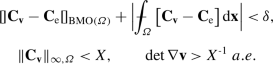(5.2)

with $${\mathbf{C}}_{\mathbf{v}}:=(\nabla {\mathbf{v}})^{\mathrm{T}}\nabla { \mathbf{v}}$$, will also satisfy

$${\mathscr {E}}({\mathbf{v}})\ge {\mathscr {E}}({\mathbf{u}}_{\mathrm{e}}) + k \int _{\varOmega }|{\mathbf{C}}_{\mathbf{v}}-{\mathbf{C}}_{\mathrm{e}}|^{2}{ \mathrm{d}}{\mathbf{x}}+ \frac{1}{2}\int _{\varOmega }{\mathbf{K}}\big({ \mathbf{x}},{\mathbf{C}}_{\mathrm{e}}({\mathbf{x}})\big):{\mathbf{H}}^{ \mathrm{T}}{\mathbf{H}}\,{\mathrm{d}}{\mathbf{x}},$$
(5.3)

where $${\mathbf{H}}:=\nabla {\mathbf{v}}-\nabla {{\mathbf{u}}_{\mathrm{e}}}$$. Moreover, if in addition $${\mathbf{v}}={\mathbf{v}}_{\mathrm{e}}$$ is a weak solution of the equilibrium equations, then

$${\mathscr {E}}({\mathbf{u}}_{\mathrm{e}})\ge {\mathscr {E}}({\mathbf{v}}_{ \mathrm{e}}) + \frac{k}{2}\int _{\varOmega }|{\mathbf{C}}_{{\mathbf{v}}_{ \mathrm{e}}}-{\mathbf{C}}_{\mathrm{e}}|^{2}{\mathrm{d}}{\mathbf{x}}+ \frac{1}{2} \int _{\varOmega }{\mathbf{K}}\big({\mathbf{x}},{\mathbf{C}}_{{ \mathbf{v}}_{\mathrm{e}}}({\mathbf{x}})\big):{\mathbf{H}}_{\mathrm{e}}^{ \mathrm{T}}{\mathbf{H}}_{\mathrm{e}}\,{\mathrm{d}}{\mathbf{x}},$$
(5.4)

where $${\mathbf{H}}_{\mathrm{e}}:=\nabla {\mathbf{v}}_{\mathrm{e}}-\nabla {{ \mathbf{u}}_{\mathrm{e}}}$$.

### Theorem 5.2

Let $$\sigma :{\overline{\varOmega }}\times \operatorname{Psym_{\mathit{n}}}\to [0,\infty )$$ satisfy (1)(3) of Hypothesis 4.1. Suppose that $${\mathbf{u}}_{\mathrm{e}}\in \operatorname{AD}$$ is a weak solution of the equilibrium equations that satisfies (5.1) and, for some $${\tau _{u}}\in {\mathbb{R}}$$, every $${\mathbf{a}}\in {\mathbb{R}}^{n}$$, and almost every $${\mathbf{x}}\in {\varOmega }$$,

$${\mathbf{a}}\cdot {\mathbf{K}}\big({\mathbf{x}},{\mathbf{C}}_{\mathrm{e}}({ \mathbf{x}})\big){\mathbf{a}}\ge 2{\tau _{u}}|{\mathbf{a}}|^{2}.$$
(5.5)

1. (a)

$${\tau _{u}}\ge 0$$; or

2. (b)

$${{\mathbf{u}}_{\mathrm{e}}}\in C^{1}({\overline{\varOmega }};{\mathbb{R}}^{n})$$ and $${\tau _{u}}\ge -k C_{M}$$, where $$C_{M}$$ is given by Proposition 2.2.

Fix $$X\in {\mathbb{R}}$$ with $$X>||{\mathbf{C}}_{\mathrm{e}}||_{\infty ,{\varOmega }}$$ and $$X^{{\text{-}}1}<\epsilon$$ (see (5.1)). Then there exists a $${\delta }={\delta }(X)>0$$ such that any $${\mathbf{v}}\in \operatorname{AD}$$, with $${\mathbf{v}}\ne {{\mathbf{u}}_{\mathrm{e}}}$$, that satisfies (5.2) will have strictly greater energy that $${{\mathbf{u}}_{\mathrm{e}}}$$. Moreover, if $${{\mathbf{u}}_{\mathrm{e}}}$$ and $${\mathbf{v}}$$ also satisfy, for some $${\tau _{v}}\in {\mathbb{R}}$$, every $${\mathbf{a}}\in {\mathbb{R}}^{n}$$, and almost every $${\mathbf{x}}\in {\varOmega }$$,

$${\mathbf{a}}\cdot \Big[{\mathbf{K}}\big({\mathbf{x}},{\mathbf{C}}_{ \mathrm{e}}({\mathbf{x}})\big)+ {\mathbf{K}}\big({\mathbf{x}},{\mathbf{C}}_{{ \mathbf{v}}}({\mathbf{x}})\big)\Big]{\mathbf{a}}\ge 2{\tau _{v}}|{ \mathbf{a}}|^{2}$$

with either

1. (i)

$${\tau _{v}}\ge 0$$; or

2. (ii)

$$2{\tau _{v}}\ge -3kC_{M}$$ and at least one of $${{\mathbf{u}}_{\mathrm{e}}}$$ and $${\mathbf{v}}$$ is contained in $$C^{1}({\overline{\varOmega }};{\mathbb{R}}^{n})$$,

then $${\mathbf{v}}$$ cannot be a weak solution of the equilibrium equations. In particular if, for $$a.e.~{\mathbf{x}}\in {\varOmega }$$, $${\mathbf{K}}({\mathbf{x}},{\mathbf{C}}_{\mathrm{e}}({\mathbf{x}}))$$ and $${\mathbf{K}}({\mathbf{x}},{\mathbf{C}}_{{\mathbf{v}}}({\mathbf{x}}))$$ are positive semidefinite, then $${\mathbf{v}}$$ cannot be a weak solution of the equilibrium equations.

### Remark 5.3

1. The inequality

$${\mathbf{B}}:{\mathbb{C}}\big({\mathbf{x}},{\mathbf{C}}_{\mathrm{e}}({ \mathbf{x}})\big)[{\mathbf{B}}] \ge 16k|{\mathbf{B}}|^{2},\ \text{ for all {\mathbf{B}}\in \operatorname{Sym_{\mathit{n}}} and a.e.~{\mathbf{x}}\in {\varOmega }},$$

implies that, for almost every $${\mathbf{x}}\in {\varOmega }$$, the function $${\mathbf{B}}\mapsto \sigma ({\mathbf{x}},{\mathbf{B}})$$ is convex in a neighborhood of $${\mathbf{C}}_{\mathrm{e}}({\mathbf{x}})$$. Gao, Neff, Roventa, and Thiel  have shown that the positivity of the principal stresses together with the assumption that, for almost every $${\mathbf{x}}\in {\varOmega }$$, $${\mathbf{C}}_{\mathrm{e}}({\mathbf{x}})$$ is a (global) point of convexity of $${\mathbf{B}}\mapsto \sigma ({\mathbf{x}},{\mathbf{B}})$$, i.e.,

$$\sigma ({\mathbf{x}},{\mathbf{B}})\ge \sigma \big({\mathbf{x}},{ \mathbf{C}}_{\mathrm{e}}({\mathbf{x}})\big) +{{\mathrm{D}}}\sigma \big({ \mathbf{x}},{\mathbf{C}}_{\mathrm{e}}({\mathbf{x}})\big):\big[{\mathbf{B}}-{ \mathbf{C}}_{\mathrm{e}}({\mathbf{x}})\big] \ \text{ for all {\mathbf{B}}\in \operatorname{Psym_{\mathit{n}}},}$$

implies that $${\mathbf{u}}_{\mathrm{e}}$$ is an absolute minimizer of the energy. 2. The conclusions of Lemma 5.1 and Theorem 5.2 are valid under slightly more general hypotheses. It is clear that (5.1) need not be satisfied by all $${\mathbf{P}}\in L^{2}({\varOmega };\operatorname{Sym_{\mathit{n}}})$$, but only by all $${\mathbf{P}}$$ that satisfy

$${\mathbf{P}}=(\nabla {\mathbf{v}})^{\mathrm{T}}\nabla {\mathbf{v}}-( \nabla {\mathbf{u}})^{\mathrm{T}}\nabla {\mathbf{u}}$$

for some $${\mathbf{u}},{\mathbf{v}}\in \operatorname{AD}$$. For information on the characterization of such mappings see, e.g., Blume  or Ciarlet and Laurent  and the references therein.

### Proof of Lemma 5.1

We suppress the variable $${\mathbf{x}}$$ for clarity of exposition. Let $${\mathbf{u}}_{\mathrm{e}}\in \operatorname{AD}$$ be a weak solution of the equilibrium equations that satisfies (5.1). Fix $$X\in {\mathbb{R}}$$ with $$X>||{\mathbf{C}}_{\mathrm{e}}||_{\infty ,{\varOmega }}$$ and $$X^{{\text{-}}1}<\epsilon$$. Define $${\mathscr {B}}\subset \operatorname{Psym_{\mathit{n}}}$$ by

$${\mathscr {B}}:= \{{\mathbf{P}}\in \operatorname{Psym_{\mathit{n}}}: X^{{\text{-}}2}< \det { \mathbf{P}},\ |{\mathbf{P}}|< X\}.$$

Let $${\mathbf{v}}\in \operatorname{AD}$$ satisfy (5.2) for some $${\delta }>0$$ to be determined. Then (4.1), (4.4), and Lemma A.1 with $${\mathbf{V}}={\mathbf{C}}_{\mathbf{v}}$$, $${\mathbf{U}}={\mathbf{C}}_{\mathrm{e}}$$, and $${\mathbf{E}}={\mathbf{C}}_{\mathbf{v}}-{\mathbf{C}}_{\mathrm{e}}$$ yield a constant $$c=c({\mathscr {B}})>0$$ such that, for $$a.e.$$ $${\mathbf{x}}\in {\varOmega }$$,

$$\sigma ({\mathbf{C}}_{\mathbf{v}})\ge \sigma ({\mathbf{C}}_{\mathrm{e}}) + \frac{1}{2} {\mathbf{E}}:{\mathbf{K}}({\mathbf{C}}_{\mathrm{e}}) +\frac{1}{8}{ \mathbf{E}}:{\mathbb{C}}({\mathbf{C}}_{\mathrm{e}})[{\mathbf{E}}] -c|{ \mathbf{E}}|^{3}.$$
(5.6)

If we now integrate (5.6) over $${\varOmega }$$ and make use of (5.1) we find that

$$\int _{\varOmega }\sigma ({\mathbf{C}}_{\mathbf{v}})\,{\mathrm{d}}{\mathbf{x}} \ge \int _{\varOmega }\sigma ({\mathbf{C}}_{\mathrm{e}})\,{\mathrm{d}}{ \mathbf{x}}+ \frac{1}{2}\int _{\varOmega }{\mathbf{E}}:{\mathbf{K}}({\mathbf{C}}_{ \mathrm{e}})\,{\mathrm{d}}{\mathbf{x}}+ 2k\int _{\varOmega }|{\mathbf{E}}|^{2}{ \mathrm{d}}{\mathbf{x}}- c\int _{\varOmega }|{\mathbf{E}}|^{3}\,{\mathrm{d}}{ \mathbf{x}}.$$
(5.7)

We next consider the term $${\mathbf{E}}:{\mathbf{K}}({\mathbf{C}}_{\mathrm{e}})$$. We make use of an observation in  (see, also, ) to write

$${\mathbf{E}}={\mathbf{C}}_{\mathbf{v}}-{\mathbf{C}}_{\mathrm{e}}= (\nabla { \mathbf{u}}_{\mathrm{e}})^{\mathrm{T}}{\mathbf{H}}+ {\mathbf{H}}^{ \mathrm{T}}\nabla {\mathbf{u}}_{\mathrm{e}}+{\mathbf{H}}^{\mathrm{T}}{ \mathbf{H}}, \qquad {\mathbf{H}}:=\nabla {\mathbf{v}}-\nabla {\mathbf{u}}_{ \mathrm{e}}.$$
(5.8)

Therefore, (4.2), (5.8), and the symmetry of $${\mathbf{K}}$$ gives us

\begin{aligned} {\mathbf{E}}:{\mathbf{K}}({\mathbf{C}}_{\mathrm{e}})&= 2{\mathbf{H}}:\big[ \nabla {\mathbf{u}}_{\mathrm{e}}{\mathbf{K}}({\mathbf{C}}_{\mathrm{e}}) \big] + {\mathbf{H}}^{\mathrm{T}}{\mathbf{H}}:{\mathbf{K}}({\mathbf{C}}_{ \mathrm{e}}) \\ &= 2{\mathbf{H}}:{\mathbf{S}}(\nabla {\mathbf{u}}_{\mathrm{e}}) + { \mathbf{H}}^{\mathrm{T}}{\mathbf{H}}:{\mathbf{K}}({\mathbf{C}}_{\mathrm{e}}) \end{aligned}

and consequently

\begin{aligned} \int _{\varOmega }{\mathbf{E}}:{\mathbf{K}}({\mathbf{C}}_{\mathrm{e}})\,{ \mathrm{d}}{\mathbf{x}}&= 2\int _{\varOmega }{\mathbf{H}}:{\mathbf{S}}( \nabla {\mathbf{u}}_{\mathrm{e}})\,{\mathrm{d}}{\mathbf{x}}+ \int _{ \varOmega }{\mathbf{H}}^{\mathrm{T}}{\mathbf{H}}:{\mathbf{K}}({\mathbf{C}}_{ \mathrm{e}})\,{\mathrm{d}}{\mathbf{x}}. \end{aligned}
(5.9)

We then combine (5.7) and (5.9) and make use of the identity (4.12) (which is a consequence of the equilibrium equations (4.10)) to conclude that

$${\mathscr {E}}({\mathbf{v}})\ge {\mathscr {E}}({\mathbf{u}}_{\mathrm{e}}) +2k \int _{\varOmega }|{\mathbf{E}}|^{2}\,{\mathrm{d}}{\mathbf{x}}- c\int _{\varOmega }|{\mathbf{E}}|^{3}\,{\mathrm{d}}{\mathbf{x}}+\frac{1}{2} \int _{ \varOmega }{\mathbf{H}}^{\mathrm{T}}{\mathbf{H}}:{\mathbf{K}}({\mathbf{C}}_{ \mathrm{e}})\,{\mathrm{d}}{\mathbf{x}}.$$
(5.10)

Next, inequality (2.7) (with $$p=2$$ and $$q=3$$) in Proposition 2.5 yields a constant $$J>0$$ such that, for the given $${\mathbf{u}}_{\mathrm{e}}$$ and $${\mathbf{v}}$$ that satisfy (5.2) and every $$i,j\in \{1,2,\ldots ,n\}$$,

$${\delta }c J^{3}\int _{\varOmega }|E_{ij}|^{2}\,{\mathrm{d}}{\mathbf{x}}\ge c \int _{\varOmega }|E_{ij}|^{3}\,{\mathrm{d}}{\mathbf{x}},$$
(5.11)

which together with (5.10) yields the desired result, (5.3), when $$\delta$$ is sufficiently small.

Finally, suppose that $${\mathbf{v}}={\mathbf{v}}_{\mathrm{e}}\not \equiv {\mathbf{u}}_{ \mathrm{e}}$$ is a solution of the equilibrium equations. Then the above argument with $${\mathbf{u}}_{\mathrm{e}}$$ replaced by $${\mathbf{v}}_{\mathrm{e}}$$ and $${\mathbf{v}}$$ replaced by $${\mathbf{u}}_{\mathrm{e}}$$ yields (see (5.6)–(5.11)) the desired inequality, (5.4), when $$\delta$$ is sufficiently small after an additional observation: Lemma 4.7 together with (5.1) implies that the constant $$k$$ in (5.3) becomes $$k/2$$ in (5.4) (see (5.6) and (5.7)). □

### Proof of Theorem 5.2

We again suppress the variable $${\mathbf{x}}$$ for clarity of exposition. Let $${\mathbf{u}}_{\mathrm{e}}\in \operatorname{AD}$$ be a weak solution of the equilibrium equations that satisfies (5.1) and (5.5). Then, in view of Lemma 4.6 (with $${\mathbf{L}}={\mathbf{K}}-2{\tau _{u}}{\mathbf{I}}$$),

$$\int _{\varOmega }{\mathbf{K}}\big({\mathbf{x}},{\mathbf{C}}_{\mathrm{e}}({ \mathbf{x}})\big):{\mathbf{H}}^{\mathrm{T}}{\mathbf{H}}\,{\mathrm{d}}{ \mathbf{x}}\ge 2{\tau _{u}}\int _{\varOmega }|{\mathbf{H}}|^{2}\,{\mathrm{d}}{ \mathbf{x}}, \qquad {\mathbf{H}}:=\nabla {\mathbf{v}}-\nabla {{\mathbf{u}}_{ \mathrm{e}}}.$$
(5.12)

Let $$\delta >0$$ be given by Lemma 5.1 so that any $${\mathbf{v}}\in \operatorname{AD}$$ that satisfies (5.2) will also satisfy (5.3), that is,

$${\mathscr {E}}({\mathbf{v}})\ge {\mathscr {E}}({\mathbf{u}}_{\mathrm{e}}) + k \int _{\varOmega }|{\mathbf{C}}_{\mathbf{v}}-{\mathbf{C}}_{\mathrm{e}}|^{2}{ \mathrm{d}}{\mathbf{x}}+ {\tau _{u}}\int _{\varOmega }|{\mathbf{H}}|^{2}\,{ \mathrm{d}}{\mathbf{x}},$$
(5.13)

where we have made use of (5.12).

(a). Clearly, $${\tau _{u}}\ge 0$$ yields $${\mathscr {E}}({\mathbf{v}})\ge {\mathscr {E}}({\mathbf{u}}_{\mathrm{e}})$$ since $$k>0$$. Suppose that $${\mathscr {E}}({\mathbf{v}})= {\mathscr {E}}({\mathbf{u}}_{\mathrm{e}})$$. Then $${\mathbf{C}}_{\mathbf{v}}={\mathbf{C}}_{\mathrm{e}}~a.e$$. Note that, in view of (5.1)2,

$$|\nabla {{\mathbf{u}}_{\mathrm{e}}}({\mathbf{x}})|^{n}\le \|\nabla {{ \mathbf{u}}_{\mathrm{e}}}\|^{n}_{\infty ,{\varOmega }} \le \bigg[ \frac{\|\nabla {{\mathbf{u}}_{\mathrm{e}}}\|^{n}_{\infty ,{\varOmega }}}{\epsilon } \bigg] \big[\det \nabla {{\mathbf{u}}_{\mathrm{e}}}({\mathbf{x}})\big] \ \text{ for a.e.~{\mathbf{x}}\in {\varOmega }.}$$

Consequently, Proposition 2.1 yields a rotation $${\mathbf{R}}\in \operatorname{SO}(n)$$ such that $$\nabla {\mathbf{v}}={\mathbf{R}}\nabla {{\mathbf{u}}_{\mathrm{e}}}~a.e$$. Since $${\varOmega }$$ is a connected open set, $${\mathbf{v}}={\mathbf{R}}{\mathbf{u}}_{\mathrm{e}}+{\mathbf{a}}$$ for some $${\mathbf{a}}\in {\mathbb{R}}^{n}$$. However, $${\mathbf{v}}={\mathbf{u}}_{\mathrm{e}}$$ on $${\mathscr {D}}$$ and so $${\mathbf{R}}={\mathbf{I}}$$ and $${\mathbf{a}}=\mathbf{0}$$ since $${\mathscr {D}}$$ is relatively open. This establishes the theorem under hypothesis (a).

(b). Suppose now that $${{\mathbf{u}}_{\mathrm{e}}}\in C^{1}({\overline{\varOmega }};{\mathbb{R}}^{n})$$ and $${\tau _{u}}\ge -k C_{M}$$, where $$C_{M}$$ is given by Proposition 2.2. Then (5.13) together with Proposition 2.2 (with $$p=q=2$$) yields

$${\mathscr {E}}({\mathbf{v}})\ge {\mathscr {E}}({\mathbf{u}}_{\mathrm{e}}) + kC_{M} \Big(\|{\mathbf{v}}-{\mathbf{u}}_{\mathrm{e}}\|_{W^{1,2}(\varOmega )}\Big)^{2} -kC_{M} \int _{\varOmega }|\nabla {\mathbf{v}}-\nabla {{\mathbf{u}}_{ \mathrm{e}}}|^{2}{\mathrm{d}}{\mathbf{x}}.$$

Thus, $${\mathbf{v}}\not \equiv {\mathbf{u}}_{\mathrm{e}}$$ satisfies $${\mathscr {E}}({\mathbf{v}})>{\mathscr {E}}({\mathbf{u}}_{\mathrm{e}})$$, as claimed.

Now suppose in addition that $${\mathbf{v}}={\mathbf{v}}_{\mathrm{e}}$$ is a weak solution of the equilibrium equations. Then (5.3) and (5.4) yield

$$0\ge 3k\int _{\varOmega }|{\mathbf{C}}_{{\mathbf{v}}_{\mathrm{e}}}-{\mathbf{C}}_{ \mathrm{e}}|^{2}{\mathrm{d}}{\mathbf{x}}+ \int _{\varOmega }\Big[{\mathbf{K}} \big({\mathbf{x}},{\mathbf{C}}_{\mathrm{e}}({\mathbf{x}})\big) +{ \mathbf{K}}\big({\mathbf{x}},{\mathbf{C}}_{{\mathbf{v}}_{\mathrm{e}}}({ \mathbf{x}})\big)\Big]:{\mathbf{H}}^{\mathrm{T}}{\mathbf{H}}\,{\mathrm{d}}{ \mathbf{x}}.$$
(5.14)

Next, in view of Lemma 4.6 (with $${\mathbf{L}}={\mathbf{K}}({\mathbf{C}}_{\mathrm{e}})+{\mathbf{K}}({ \mathbf{C}}_{{\mathbf{v}}_{\mathrm{e}}})-2{\tau _{v}}{\mathbf{I}}$$)

$$\int _{\varOmega }\Big[{\mathbf{K}}\big({\mathbf{x}},{\mathbf{C}}_{\mathrm{e}}({ \mathbf{x}})\big) +{\mathbf{K}}\big({\mathbf{x}},{\mathbf{C}}_{{\mathbf{v}}_{ \mathrm{e}}}({\mathbf{x}})\big)\Big]:{\mathbf{H}}^{\mathrm{T}}{\mathbf{H}} \,{\mathrm{d}}{\mathbf{x}}\ge 2{\tau _{v}}\int _{\varOmega }|{\mathbf{H}}|^{2} \,{\mathrm{d}}{\mathbf{x}},$$

which together with (5.14) gives us

$$0\ge 3k\int _{\varOmega }|{\mathbf{C}}_{{\mathbf{v}}_{\mathrm{e}}}-{\mathbf{C}}_{ \mathrm{e}}|^{2}{\mathrm{d}}{\mathbf{x}}+2{\tau _{v}}\int _{\varOmega }| \nabla {\mathbf{v}}_{\mathrm{e}}-\nabla {{\mathbf{u}}_{\mathrm{e}}}|^{2}{ \mathrm{d}}{\mathbf{x}}.$$
(5.15)

(i). If $${\tau _{v}}\ge 0$$, then $$k>0$$ yields $${\mathbf{C}}_{{\mathbf{v}}_{\mathrm{e}}}={\mathbf{C}}_{\mathrm{e}}~a.e$$. and the same argument used to prove (a) now yields $${\mathbf{v}}_{\mathrm{e}}={{\mathbf{u}}_{\mathrm{e}}}$$. Thus, $${\mathbf{v}}\not \equiv {\mathbf{u}}_{\mathrm{e}}$$ cannot satisfy the equilibrium equations.

(ii). Suppose that $$2{\tau _{v}}\ge -3kC_{M}$$ and at least one of $${{\mathbf{u}}_{\mathrm{e}}}$$ and $${\mathbf{v}}$$ is contained in $$C^{1}({\overline{\varOmega }};{\mathbb{R}}^{n})$$. Then (5.15) together with Proposition 2.2 (with $$p=q=2$$) now yields

$$0\ge 3k C_{M}\Big(\|{\mathbf{v}}_{\mathrm{e}}-{\mathbf{u}}_{\mathrm{e}}\|_{W^{1,2}( \varOmega )}\Big)^{2} -3kC_{M} \int _{\varOmega }|\nabla {\mathbf{v}}_{ \mathrm{e}}-\nabla {{\mathbf{u}}_{\mathrm{e}}}|^{2}{\mathrm{d}}{\mathbf{x}}.$$

Therefore, $${\mathbf{v}}_{\mathrm{e}}={{\mathbf{u}}_{\mathrm{e}}}$$ and hence $${\mathbf{v}}\not \equiv {\mathbf{u}}_{\mathrm{e}}$$ cannot satisfy the equilibrium equations. □

### Deformations with Small Strain

In this subsection we focus on deformations $${\mathbf{u}}\in \operatorname{AD}$$ whose nonlinear Green-St. Venant strain tensor

$${\mathbf{E}}_{\mathbf{u}}({\mathbf{x}}):= \frac{1}{2}\big[{\mathbf{C}}_{ \mathbf{u}}({\mathbf{x}})-{\mathbf{I}}\big]$$
(5.16)

is sufficiently small. Given one equilibrium solution $${{\mathbf{u}}_{\mathrm{e}}}$$ whose strain tensor $${\mathbf{E}}_{\mathrm{e}}$$ is uniformly and sufficiently small we apply Theorem 5.2 to show that there is a $$\operatorname{BMO}\cap \,L^{1}$$ neighborhood in strain space where there are no other solutions of the equilibrium equations.

### Corollary 5.4

Let $$\sigma$$ satisfy (1)(3) of Hypothesis 4.1. Suppose that, for some $$k>0$$,

$${\mathbf{B}}:{\mathbb{C}}({\mathbf{x}},{\mathbf{I}})[{\mathbf{B}}]\ge 32k |{ \mathbf{B}}|^{2},$$

for every $${\mathbf{B}}\in \operatorname{Sym_{\mathit{n}}}$$ and $$a.e.~{\mathbf{x}}\in \varOmega$$. Assume further that $${\mathbf{u}}_{\mathrm{e}}\in \operatorname{AD}$$ is a weak solution of the equilibrium equations that satisfies, for some $${\tau _{u}}\in {\mathbb{R}}$$, some $$\epsilon \in (0,1)$$, every $${\mathbf{a}}\in {\mathbb{R}}^{n}$$, and almost every $${\mathbf{x}}\in {\varOmega }$$,

$$\begin{gathered} {\mathbf{a}}\cdot {\mathbf{K}}\big({\mathbf{x}},{\mathbf{C}}_{\mathrm{e}}({ \mathbf{x}})\big){\mathbf{a}}\ge 2{\tau _{u}}|{\mathbf{a}}|^{2}, \qquad \det \nabla {\mathbf{u}}_{\mathrm{e}}({\mathbf{x}})> \epsilon , \qquad \big\| {\mathbf{C}}_{\mathrm{e}}-{\mathbf{I}}\big\| _{\infty ,\varOmega } < \omega _{\mathrm{o}}, \end{gathered}$$
(5.17)

where $${\mathbf{C}}_{\mathrm{e}}={\mathbf{C}}_{{\mathbf{u}}_{\mathrm{e}}}:=( \nabla {\mathbf{u}}_{\mathrm{e}})^{\mathrm{T}}\nabla {\mathbf{u}}_{ \mathrm{e}}$$, $$\omega _{\mathrm{o}}$$ is the constant determined in Lemma 4.8 (with $${\mathbf{C}}_{\mathrm{o}}={\mathbf{I}}$$), and either

1. (a)

$${\tau _{u}}\ge 0$$; or

2. (b)

$${{\mathbf{u}}_{\mathrm{e}}}\in C^{1}({\overline{\varOmega }};{\mathbb{R}}^{n})$$ and $${\tau _{u}}\ge -k C_{M}$$, where $$C_{M}$$ is given by Proposition 2.2.

Fix $$X\in {\mathbb{R}}$$ with $$X>||{\mathbf{C}}_{\mathrm{e}}||_{\infty ,{\varOmega }}$$ and $$X^{{\text{-}}1}<\epsilon$$ and suppose that $${\mathbf{v}}\in \operatorname{AD}$$ satisfies

$$||{\mathbf{C}}_{\mathbf{v}}||_{\infty ,{\varOmega }} < X, \qquad \det \nabla {\mathbf{v}}>X^{{\text{-}}1}~a.e.,$$

with $${\mathbf{C}}_{\mathbf{v}}:=(\nabla {\mathbf{v}})^{\mathrm{T}}\nabla { \mathbf{v}}$$. Then there exists a $${\delta }={\delta }(X)>0$$ such that if $${\mathbf{u}}_{\mathrm{e}}$$ and $${\mathbf{v}}$$ satisfy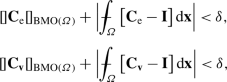(5.18)

or, merely,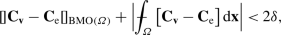(5.19)

then $${\mathbf{v}}\ne {{\mathbf{u}}_{\mathrm{e}}}$$ will have strictly greater energy that $${{\mathbf{u}}_{\mathrm{e}}}$$. Moreover, if $${{\mathbf{u}}_{\mathrm{e}}}$$ and $${\mathbf{v}}$$ also satisfy, for some $${\tau _{v}}\in {\mathbb{R}}$$, every $${\mathbf{a}}\in {\mathbb{R}}^{n}$$, and almost every $${\mathbf{x}}\in {\varOmega }$$,

$${\mathbf{a}}\cdot \Big[{\mathbf{K}}\big({\mathbf{x}},{\mathbf{C}}_{ \mathrm{e}}({\mathbf{x}})\big)+ {\mathbf{K}}\big({\mathbf{x}},{\mathbf{C}}_{{ \mathbf{v}}}({\mathbf{x}})\big)\Big]{\mathbf{a}}\ge 2{\tau _{v}}|{ \mathbf{a}}|^{2}$$

with either

1. (i)

$${\tau _{v}}\ge 0$$; or

2. (ii)

$$2{\tau _{v}}\ge -3kC_{M}$$ and at least one of $${{\mathbf{u}}_{\mathrm{e}}}$$ and $${\mathbf{v}}$$ is contained in $$C^{1}({\overline{\varOmega }};{\mathbb{R}}^{n})$$,

then $${\mathbf{v}}$$ cannot be a weak solution of the equilibrium equations. In particular if, for $$a.e.$$ $${\mathbf{x}}\in {\varOmega }$$, $${\mathbf{K}}({\mathbf{x}},{\mathbf{C}}_{\mathrm{e}}({\mathbf{x}}))$$ and $${\mathbf{K}}({\mathbf{x}},{\mathbf{C}}_{{\mathbf{v}}}({\mathbf{x}}))$$ are positive semidefinite, then $${\mathbf{v}}$$ cannot be a weak solution of the equilibrium equations.

### Remark 5.5

1. A simple computation shows that, for any $${\mathbf{H}}\in {\mathbb{M}}^{n\times n}$$,

$${[\kern -0.3ex] {\mathbf {C}}_{{\mathbf {v}}} -{\mathbf {H}}[\kern -0.3ex]}_{\operatorname{BMO}(\varOmega )}= {[\kern -0.3ex] {\mathbf {C}}_{{\mathbf {v}}} [\kern -0.3ex]}_{\operatorname{BMO}(\varOmega )}.$$

Thus, e.g., (5.18)2 is the assumption that $${\mathbf{C}}_{\mathbf{v}}$$ is close to the identity in $$\operatorname{BMO}\cap \,L^{1}$$. 2. Note that Corollary 5.4 does not require a stress-free reference configuration. 3. Although hypothesis (5.17)3 forces $${\mathbf{C}}_{\mathrm{e}}$$ to lie in an $$L^{\infty }$$-neighborhood of $${\mathbf{I}}$$, results of  show that $$\nabla {{\mathbf{u}}_{\mathrm{e}}}$$ then lies in a $$\operatorname{BMO}\cap \,L^{1}$$-neighborhood of some rotation. More preciselyFootnote 10 there is a constant $$D=D({\varOmega })$$ such that for any $${\mathbf{u}}\in \operatorname{AD}$$ there exists a rotation $${\mathbf{Q}}_{\mathbf{u}}\in \operatorname{SO}(n)$$ such that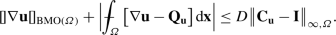### Remark 5.6

Uniqueness of equilibrium in a $$\operatorname{BMO}$$-neighborhood of a stress-free reference configuration was obtained by John  for the pure-displacement problem (see also ). That result was recently extended to the mixed problem in . The object that is small in $$\operatorname{BMO}$$ in these papers is the deformation gradient $$\nabla {\mathbf{u}}$$, rather than the strain $${\mathbf{C}}_{\mathbf{u}}$$. Thus, the neighborhood in which there are no other solutions is larger in Corollary 5.4 than in prior results. However, our result requires the additional assumption that each equilibria experience either tension or, at least, compressions that are sufficiently small.

### Proof of Corollary 5.4

We first note that the triangle inequality together with (5.18) yields (5.19). Thus we will assume that $${\mathbf{u}}_{\mathrm{e}}$$ and $${\mathbf{v}}$$ satisfy (5.19). We next observe that Lemma 4.8 yields an $$\omega _{\mathrm{o}}>0$$ such that any $${\mathbf{E}}\in \operatorname{Psym_{\mathit{n}}}$$ with $$|{\mathbf{E}}-{\mathbf{I}}|<\omega _{\mathrm{o}}$$ will satisfy

$${\mathbf{B}}:{\mathbb{C}}({\mathbf{x}},{\mathbf{E}})[{\mathbf{B}}]\ge 16k|{ \mathbf{B}}|^{2},$$
(5.20)

for all $${\mathbf{B}}\in \operatorname{Sym_{\mathit{n}}}$$ and $$a.e.~{\mathbf{x}}\in {\varOmega }$$. Consequently, (5.17)3 (together with (5.20)) yields

$$\int _{\varOmega }{\mathbf{P}}({\mathbf{x}}):{\mathbb{C}}\big({\mathbf{x}},{ \mathbf{C}}_{\mathrm{e}}({\mathbf{x}})\big) \big[{\mathbf{P}}({\mathbf{x}}) \big]\,{\mathrm{d}}{\mathbf{x}}\ge 16k\int _{\varOmega }|{\mathbf{P}}({ \mathbf{x}})|^{2}{\mathrm{d}}{\mathbf{x}},$$

for all $${\mathbf{P}}\in L^{2}({\varOmega };\operatorname{Sym_{\mathit{n}}})$$. Finally, we see that the hypotheses of Theorem 5.2 are satisfied, which then implies the desired results. □

## Reference Configurations at Equilibrium

We here note that the statement of Theorem 5.2 simplifies when the body in its reference configuration is itself at equilibrium. Thus, we assume that $${{\mathbf{u}}_{\mathrm{e}}}={\mathbf{id}}\in C^{1}({ \overline{\varOmega }};{\mathbb{R}}^{n})$$, i.e.,

$${{\mathbf{u}}_{\mathrm{e}}}({\mathbf{x}})={\mathbf{id}}({\mathbf{x}}) := { \mathbf{x}}\ \text{ for } {\mathbf{x}}\in {\varOmega },$$

where $${\varOmega }\subset {\mathbb{R}}^{n}$$ is a Lipschitz domain. Clearly, we also require that $${\mathbf{d}}={\mathbf{id}}$$ on $${\mathscr {D}}$$. However, we do not require that this reference configuration be stress free.

### Remark 6.1

The above assumption is akin to assuming that one is given a body, $$\overline{{\mathscr {B}}}\subset {\mathbb{R}}^{n}$$, and a mapping $${\mathbf{u}}:\overline{{\mathscr {B}}}\to {\mathbb{R}}^{n}$$ that is a solution of the equilibrium equations and for which the deformed body is a Lipschitz (or John) domain. However, without further assumptions on $${\mathbf{u}}$$ the two approaches are not equivalent. In particular, $${\mathbf{u}}(\partial {\mathscr {B}})$$ need not be equal to $$\partial {\varOmega }$$.

### Theorem 6.2

(Theorem 5.2 for a Reference Configuration at Equilibrium) Let $$\sigma :{\overline{\varOmega }}\times \operatorname{Psym_{\mathit{n}}}\to [0,\infty )$$ satisfy (1)(3) of Hypothesis 4.1. Suppose that $${\mathbf{u}}_{\mathrm{e}}={\mathbf{id}}$$ is a weak solution of the equilibrium equations, (4.10), that satisfies, for some $$k>0$$, every $${\mathbf{P}}\in L^{2}({\varOmega };\operatorname{Sym_{\mathit{n}}})$$, every $${\mathbf{a}}\in {\mathbb{R}}^{n}$$, and almost every $${\mathbf{x}}\in {\varOmega }$$,

$$\int _{\varOmega }{\mathbf{P}}({\mathbf{x}}):{\mathbb{C}}\big({\mathbf{x}},{ \mathbf{I}}\big) \big[{\mathbf{P}}({\mathbf{x}})\big]\,{\mathrm{d}}{ \mathbf{x}}\ge 16k\int _{\varOmega }|{\mathbf{P}}({\mathbf{x}})|^{2}{ \mathrm{d}}{\mathbf{x}},\qquad {\mathbf{a}}\cdot {\mathbf{K}}({\mathbf{x}},{ \mathbf{I}}){\mathbf{a}}\ge -2kC_{M} |{\mathbf{a}}|^{2}.$$

Fix $$X>\sqrt{n}$$. Then there exists a $${\delta }={\delta }(X)>0$$ such that any $${\mathbf{v}}\in \operatorname{AD}$$ that satisfies $${\mathbf{v}}\ne {\mathbf{id}}$$,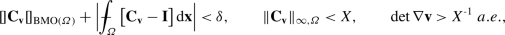with $${\mathbf{C}}_{\mathbf{v}}:=(\nabla {\mathbf{v}})^{\mathrm{T}}\nabla { \mathbf{v}}$$, will have strictly greater energy than $${\mathbf{id}}$$. Moreover, if in addition, for almost every $${\mathbf{x}}\in {\varOmega }$$ and every $${\mathbf{a}}\in {\mathbb{R}}^{n}$$,

$${\mathbf{a}}\cdot \Big[{\mathbf{K}}\big({\mathbf{x}},{\mathbf{I}}\big)+ { \mathbf{K}}\big({\mathbf{x}},{\mathbf{C}}_{{\mathbf{v}}}({\mathbf{x}}) \big)\Big]{\mathbf{a}}\ge -3kC_{M} |{\mathbf{a}}|^{2}$$

then $${\mathbf{v}}$$ cannot be a weak solution of the equations of equilibrium. In particular if, for $$a.e.~{\mathbf{x}}\in {\varOmega }$$, $${\mathbf{K}}({\mathbf{x}},{\mathbf{I}})$$ and $${\mathbf{K}}({\mathbf{x}},{\mathbf{C}}_{{\mathbf{v}}}({\mathbf{x}}))$$ are positive semidefinite, then $${\mathbf{v}}$$ cannot be a weak solution of the equilibrium equations.

### Remark 6.3

(1). A slightly better result can be obtained by replacing Proposition 2.2 with the result it is based upon in . (2). We note that statements of prior results of ours from  as well as a prior result of J. Sivaloganathan and one of the current authors from  also simplify in the special case when $${{\mathbf{u}}_{\mathrm{e}}}={\mathbf{id}}$$.